# NCERT Exemplar Class 10 Science Solutions for Chapter 3 - Metals And Non Metals

## NCERT Exemplar Solutions Class 10 Science Chapter 3 – Free PDF Download

NCERT Exemplar for Class 10 Science Chapter 3 Metals and Non-metals is available here for free download. These solutions will enable students to develop a clear understanding of important topics and questions. Students can also go through the answers provided, and once they get acquainted with the NCERT Exemplar, they will be able to study productively and even perform well in the exams. NCERT Exemplar Class 10 Science Chapter 3 available here in PDF for easy access and download.

Chemistry students already know that elements are classified as metals and non-metals. In Class 10 Science textbook, they will explore the topic in more detail in Chapter 3. They will learn about the physical as well as the chemical properties of metals and non-metals, extraction methods, the main differences between the two, recognize and classify chemical reactions or changes.

### Download PDF of NCERT exemplar for Class 10 Science Chapter 3 – Metals and Non-metals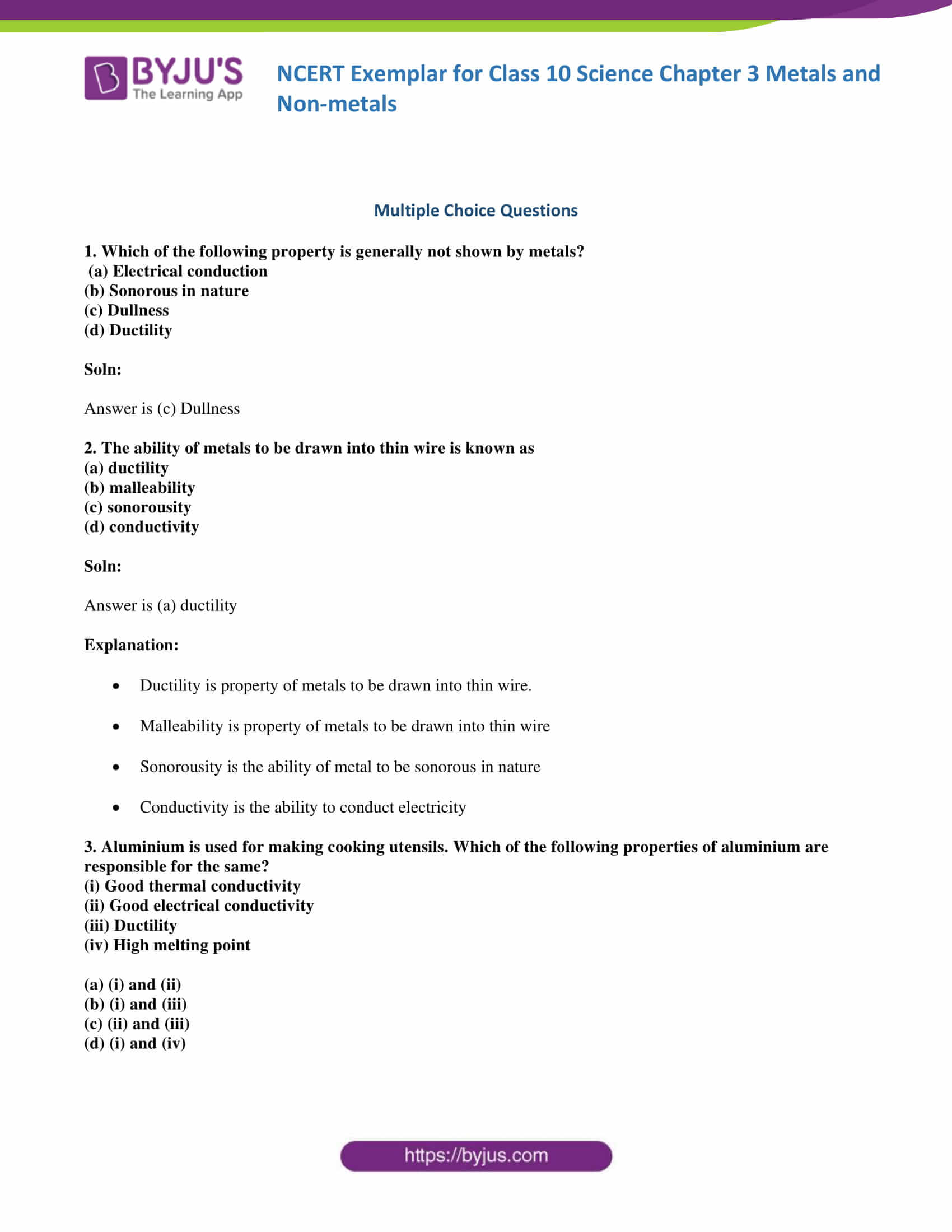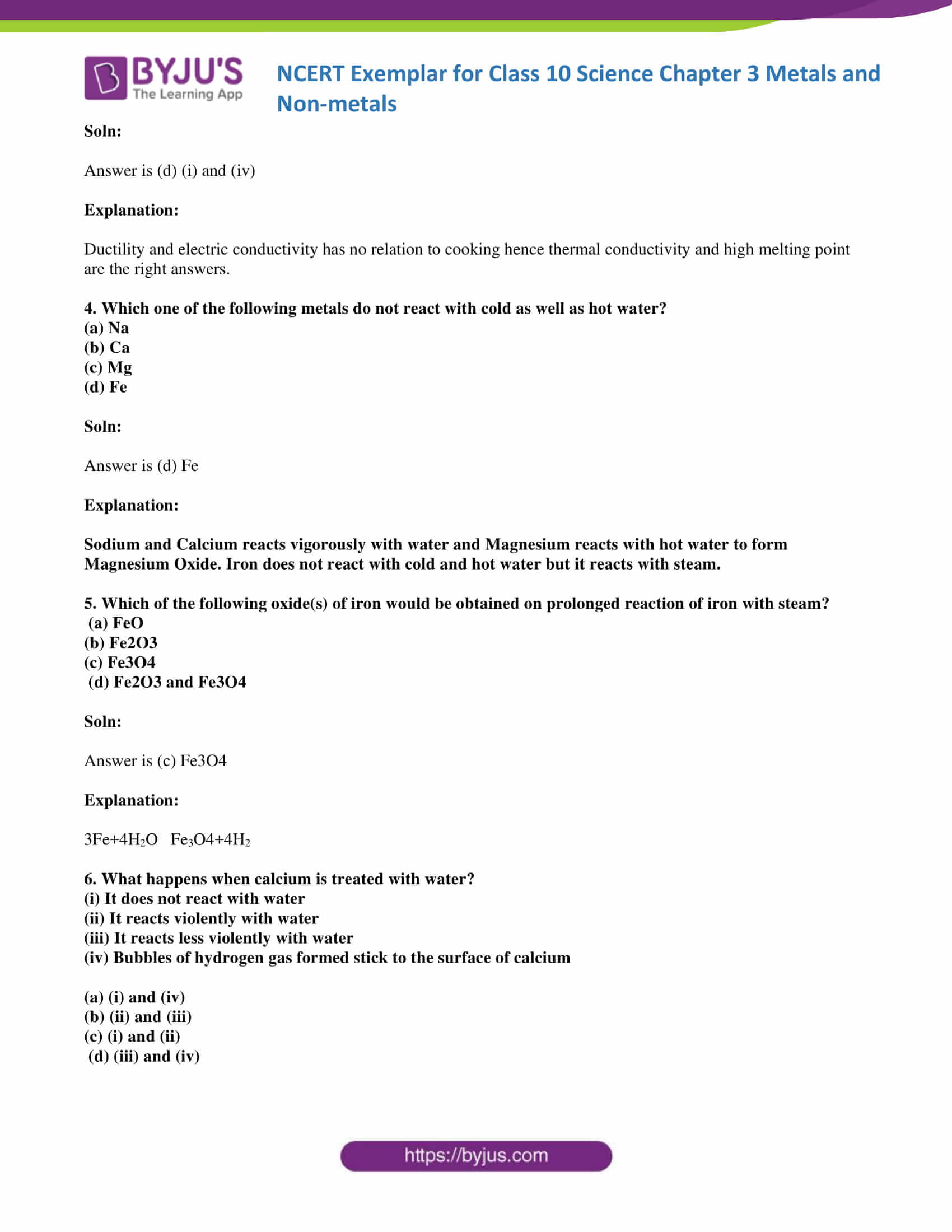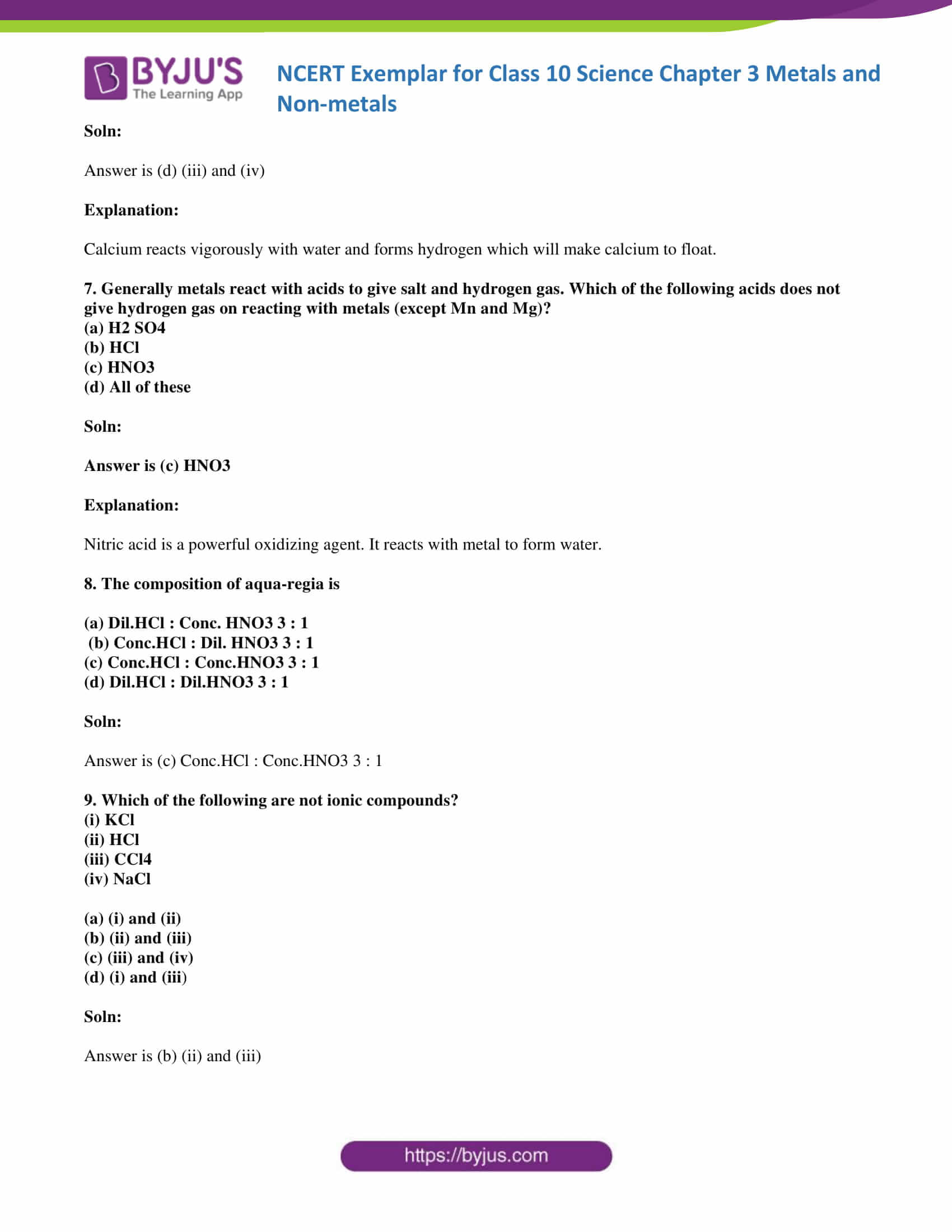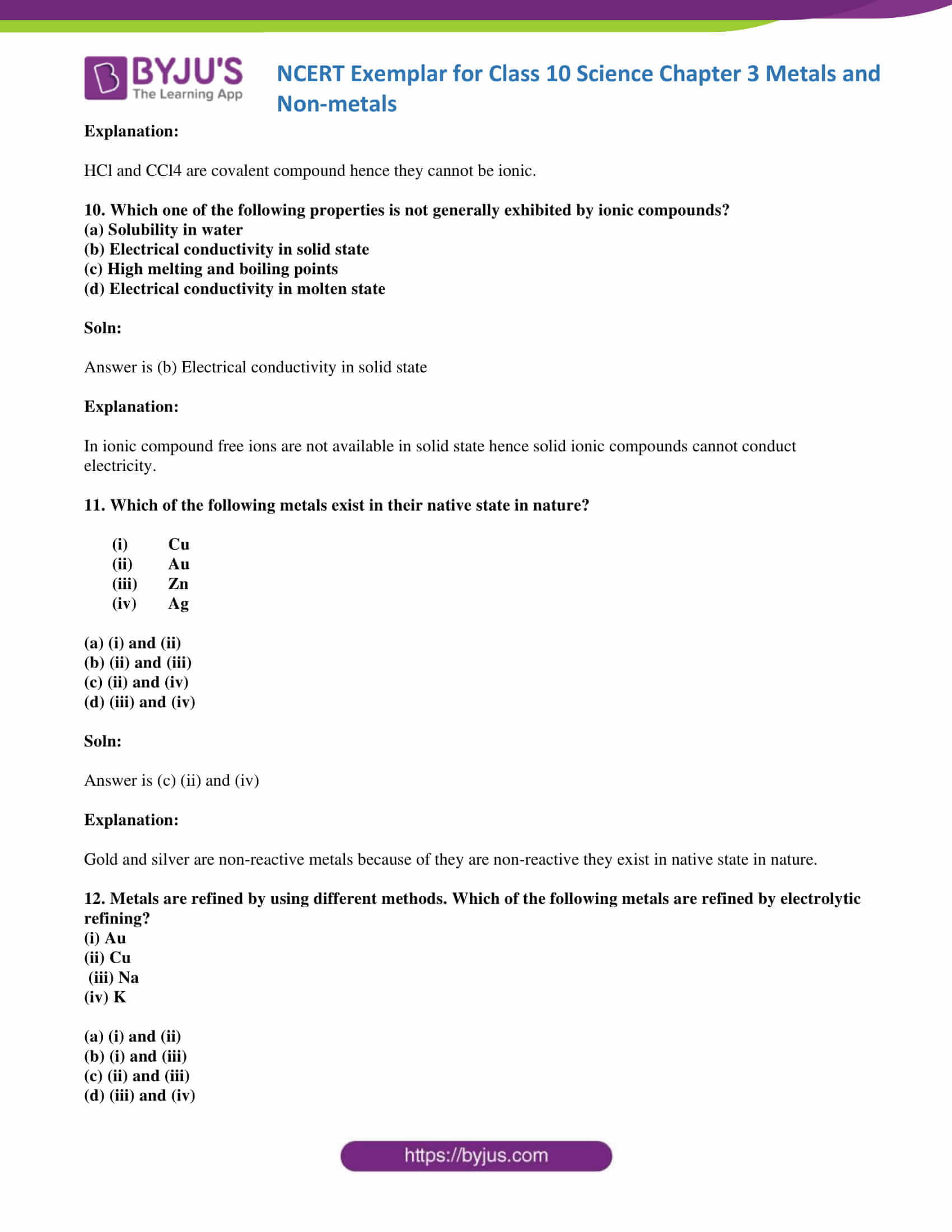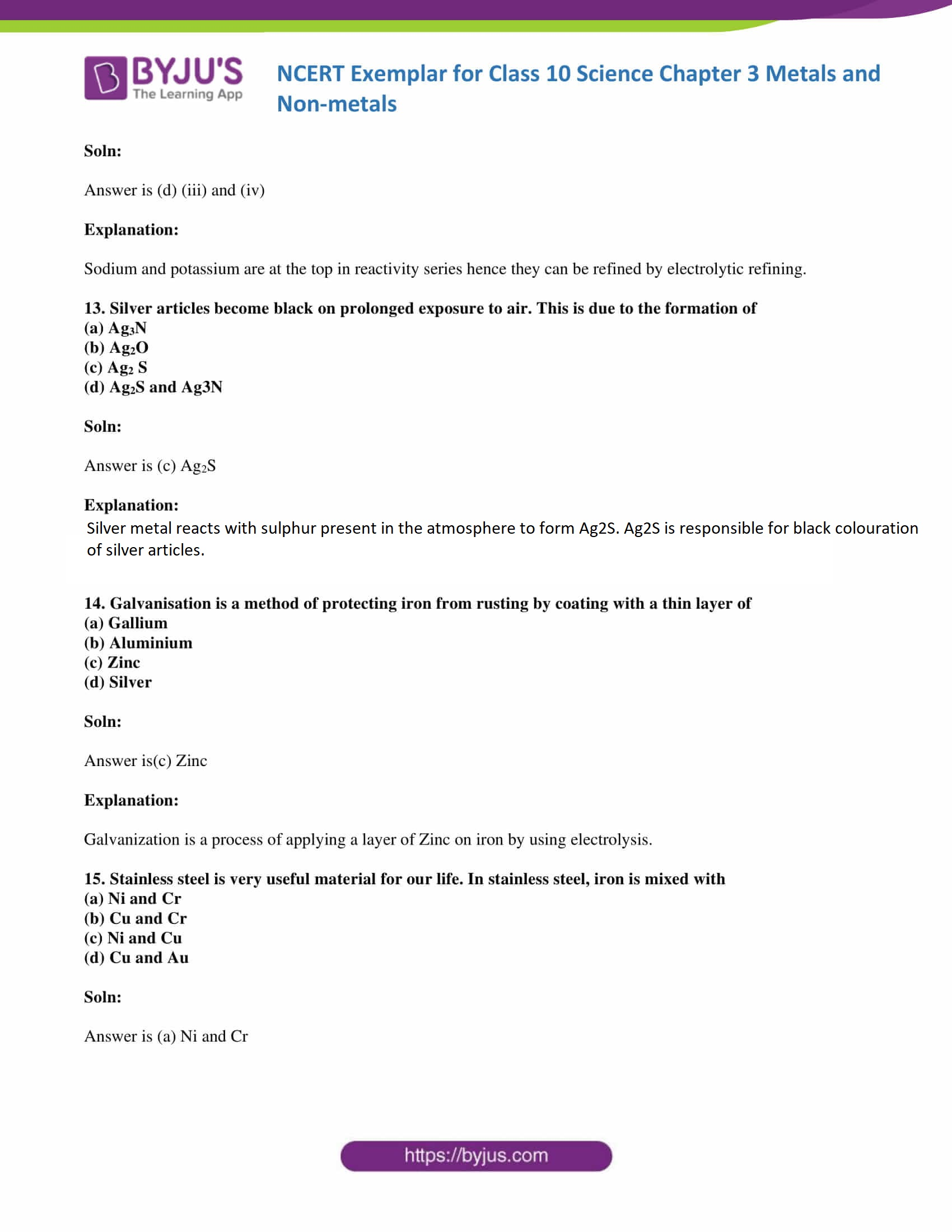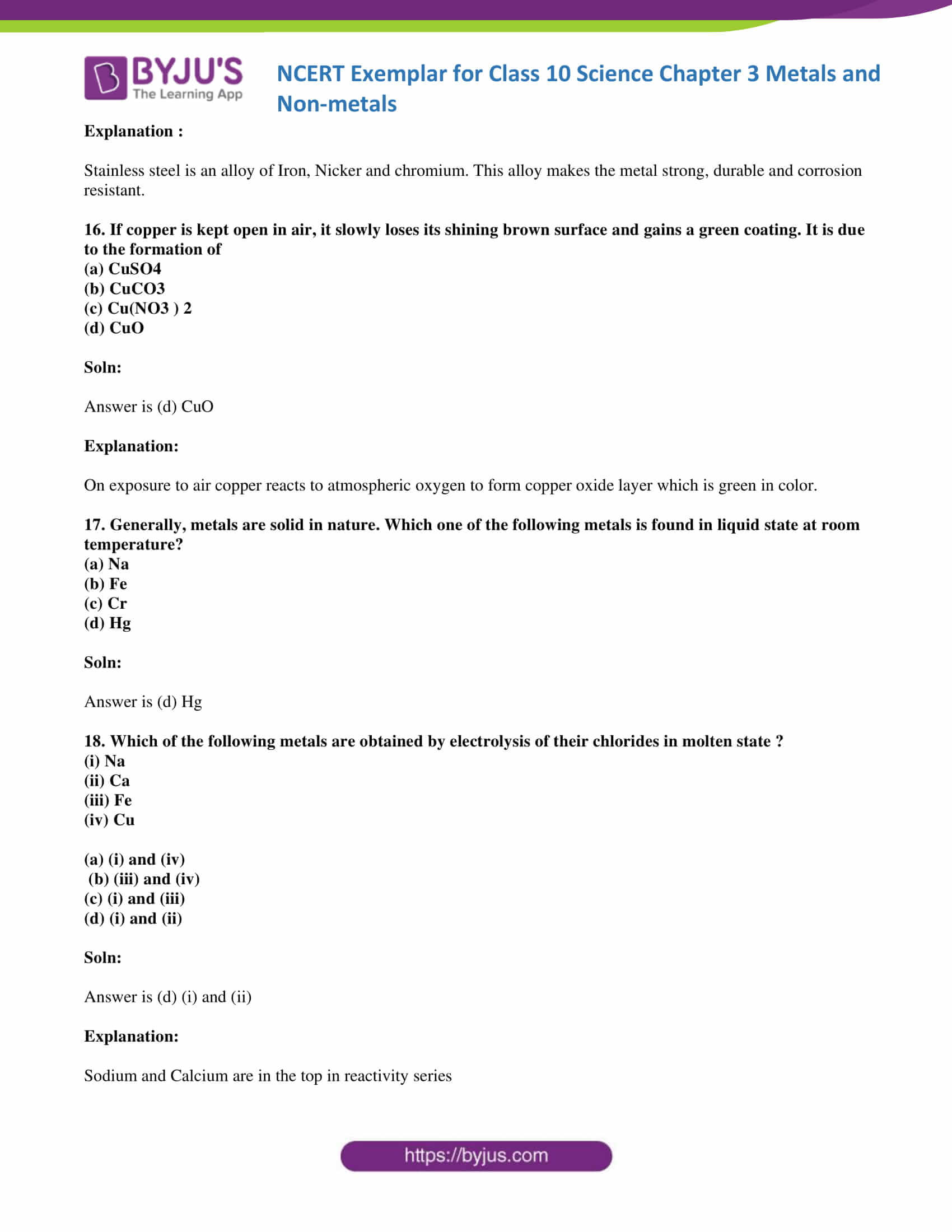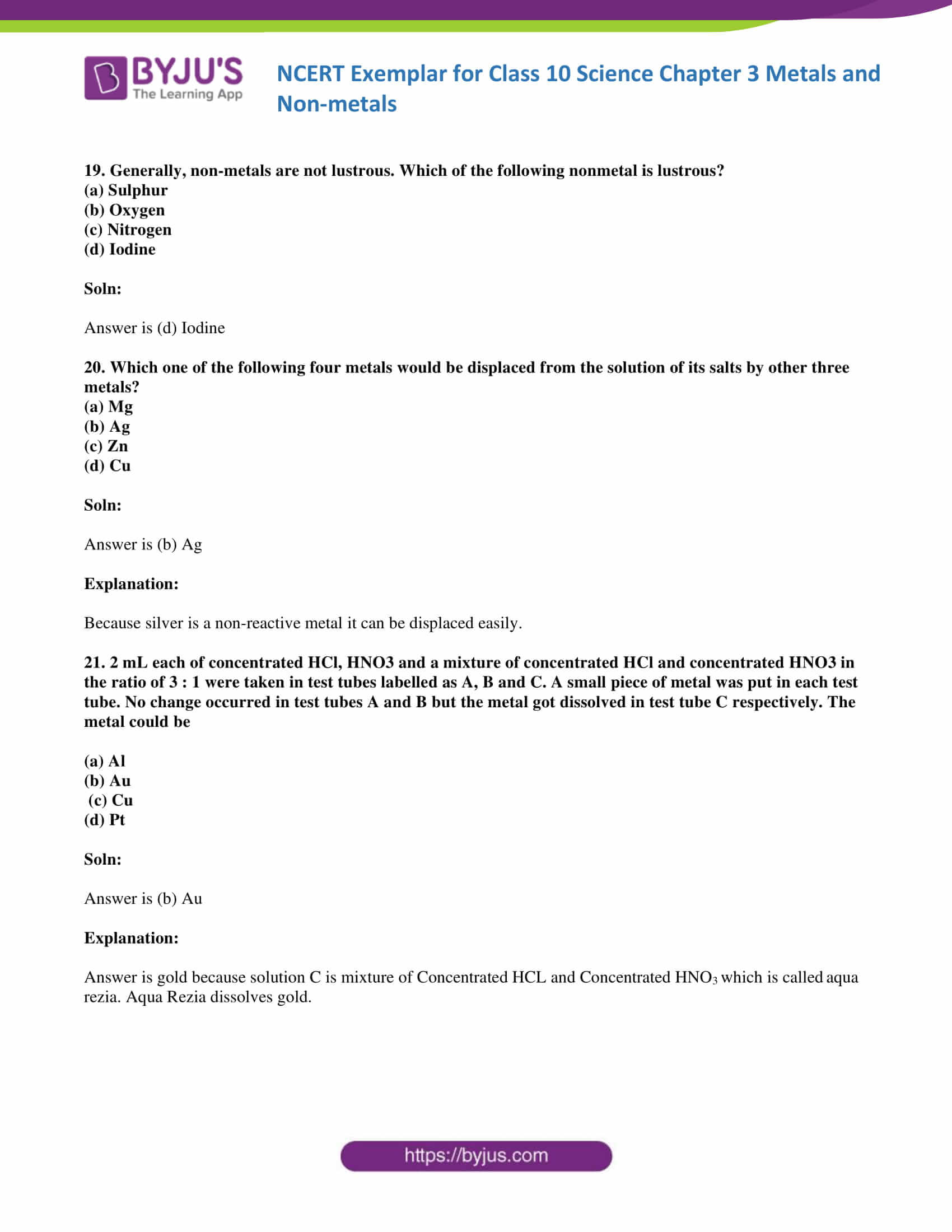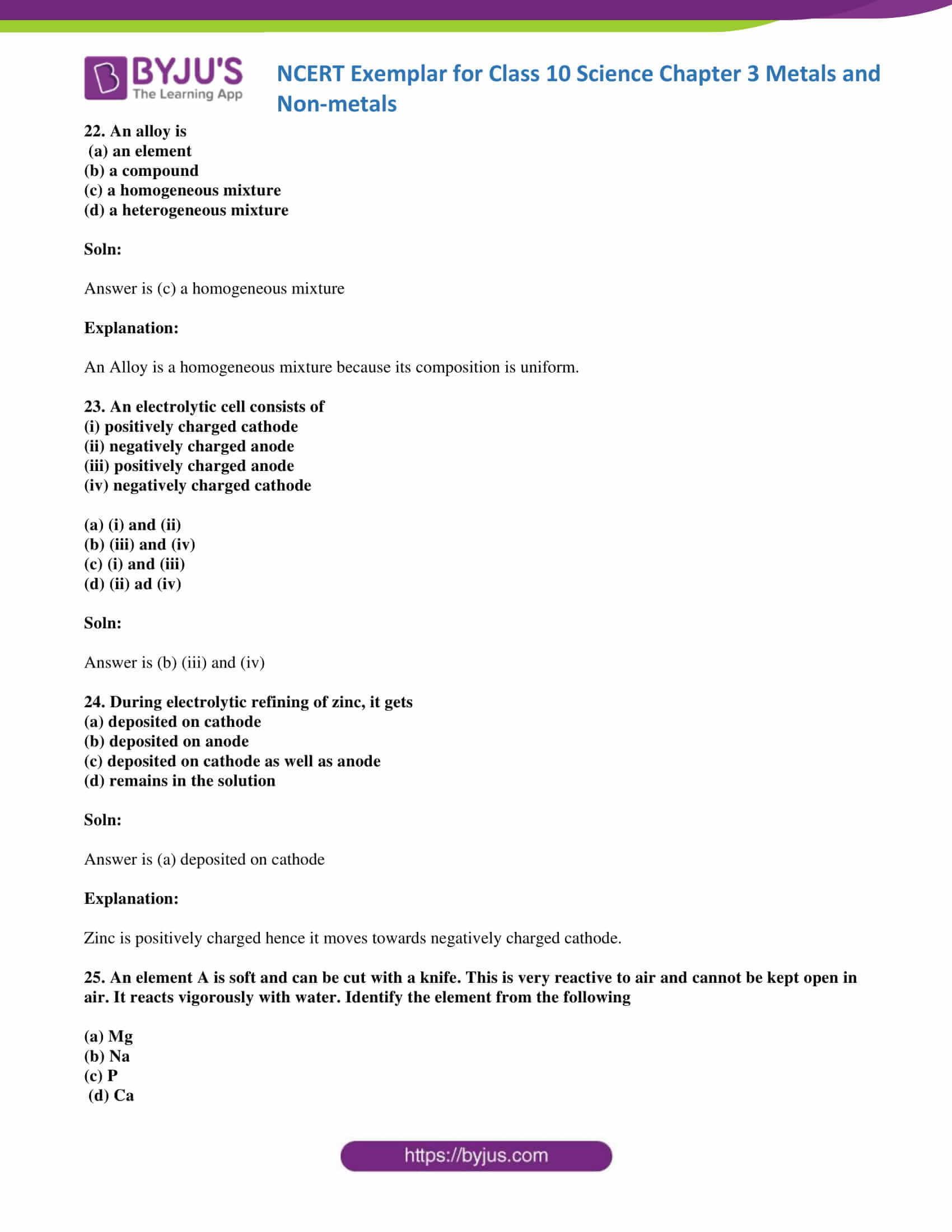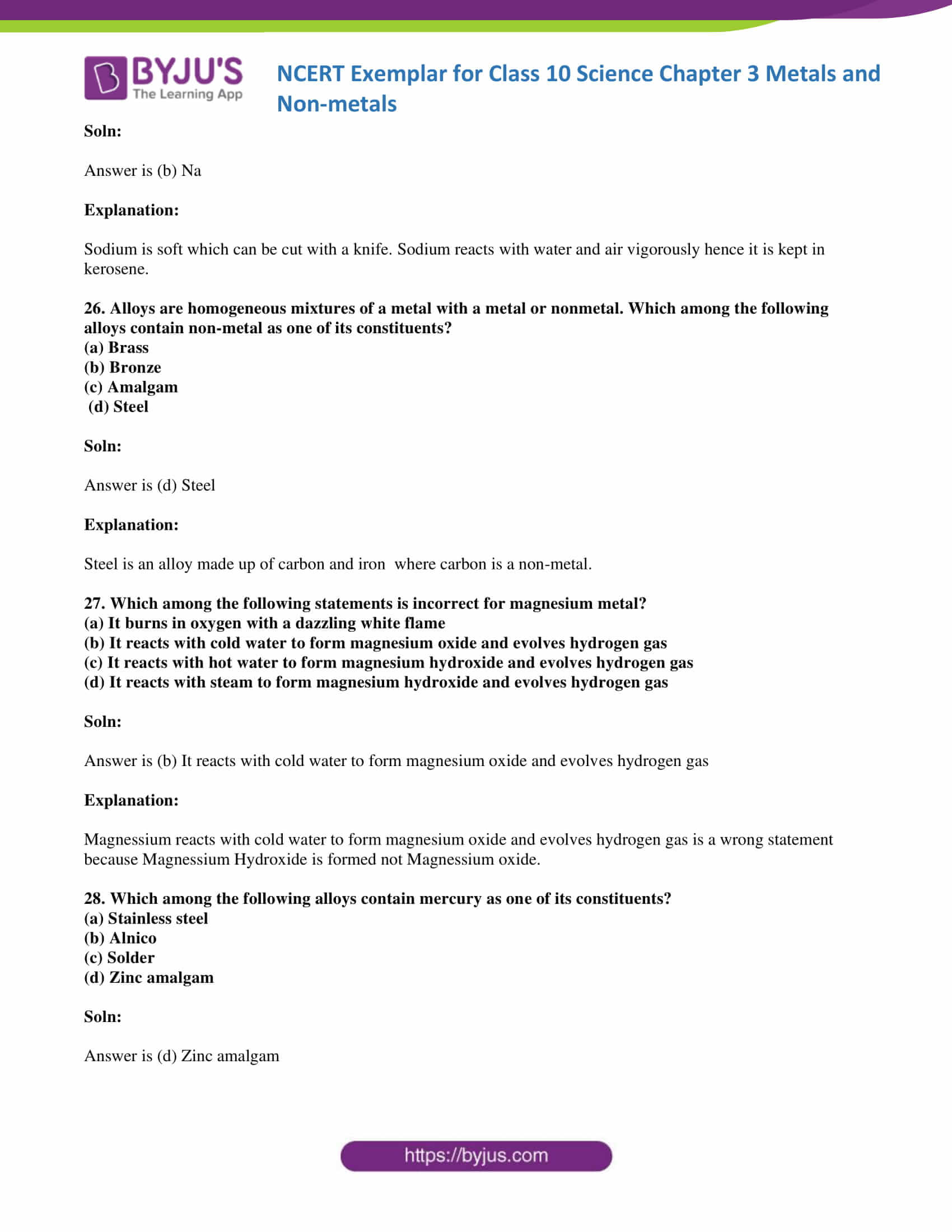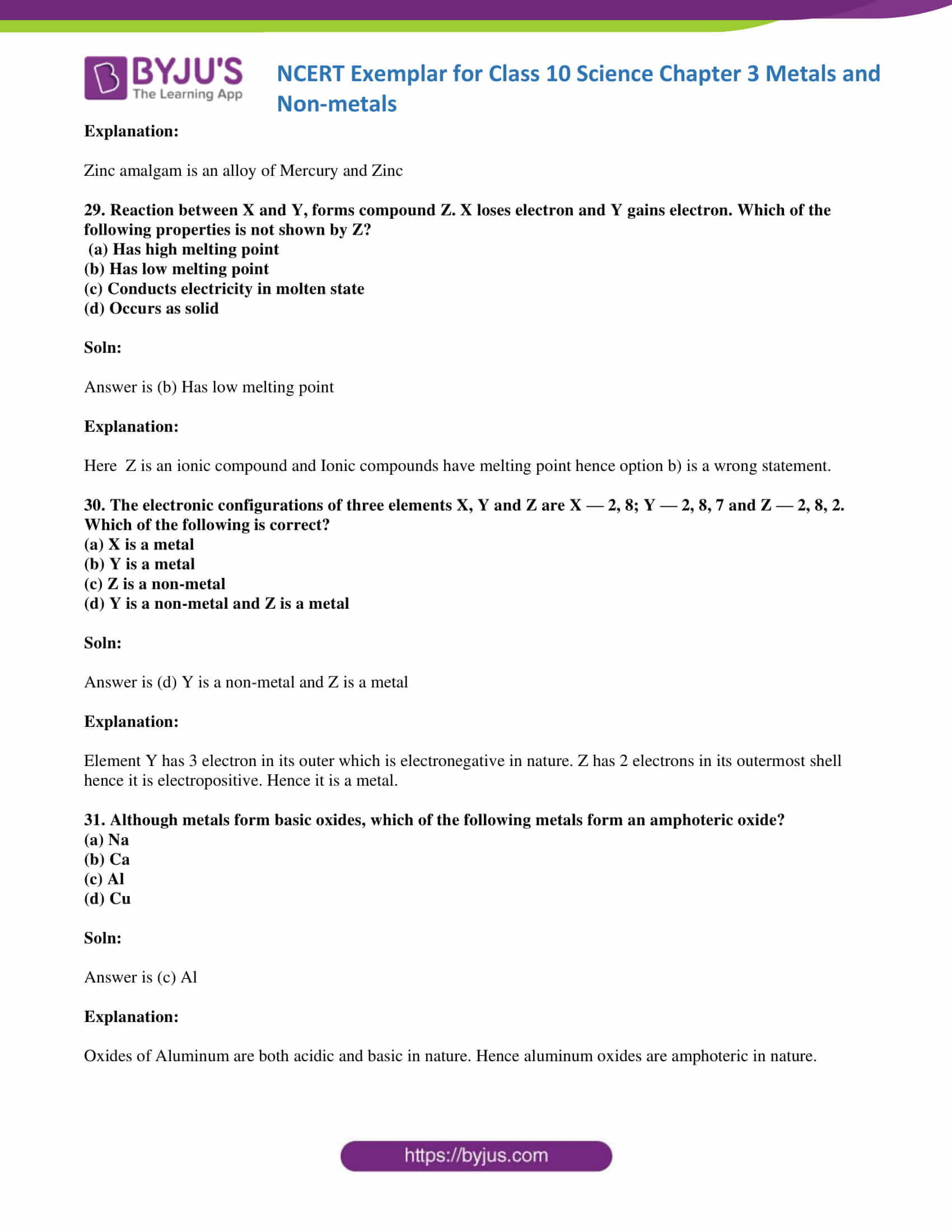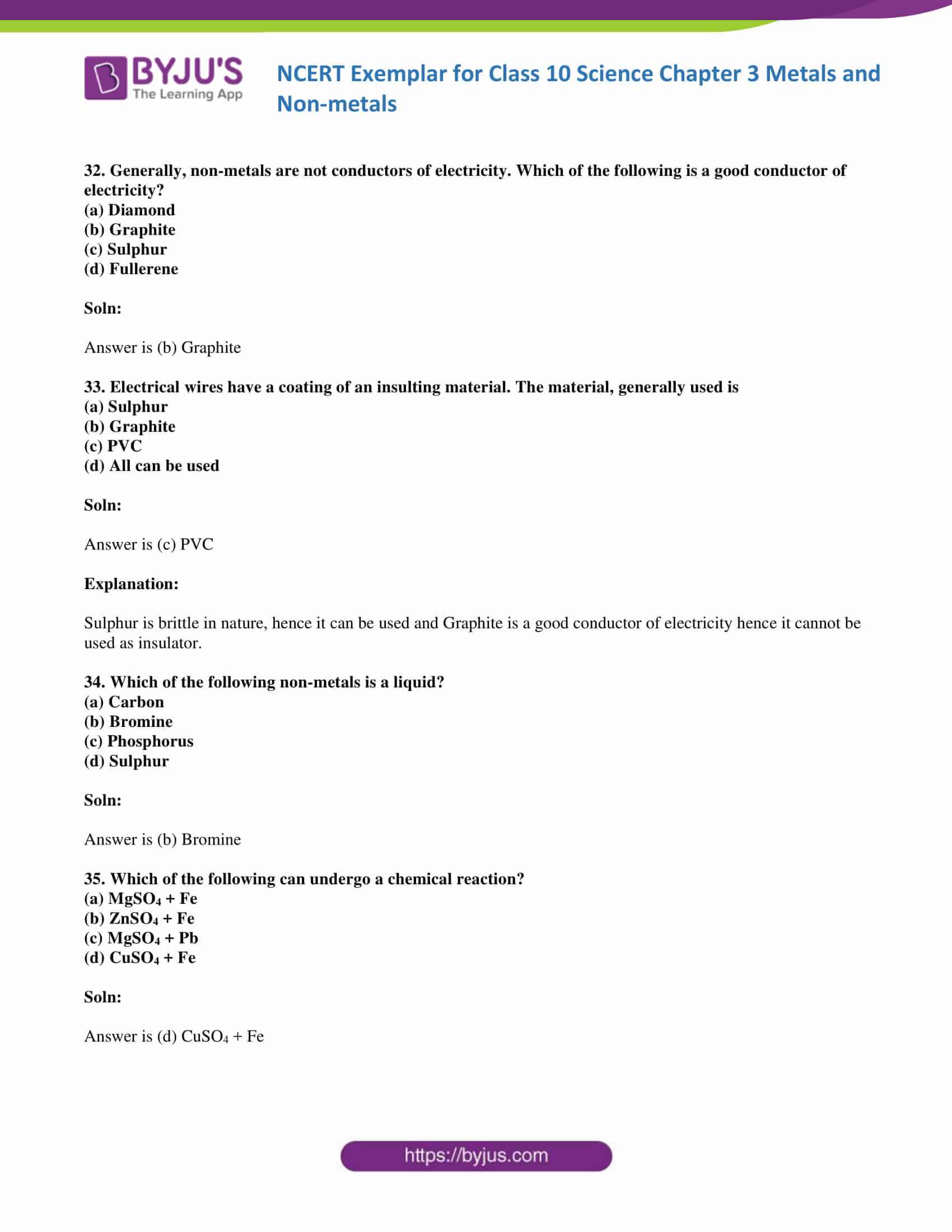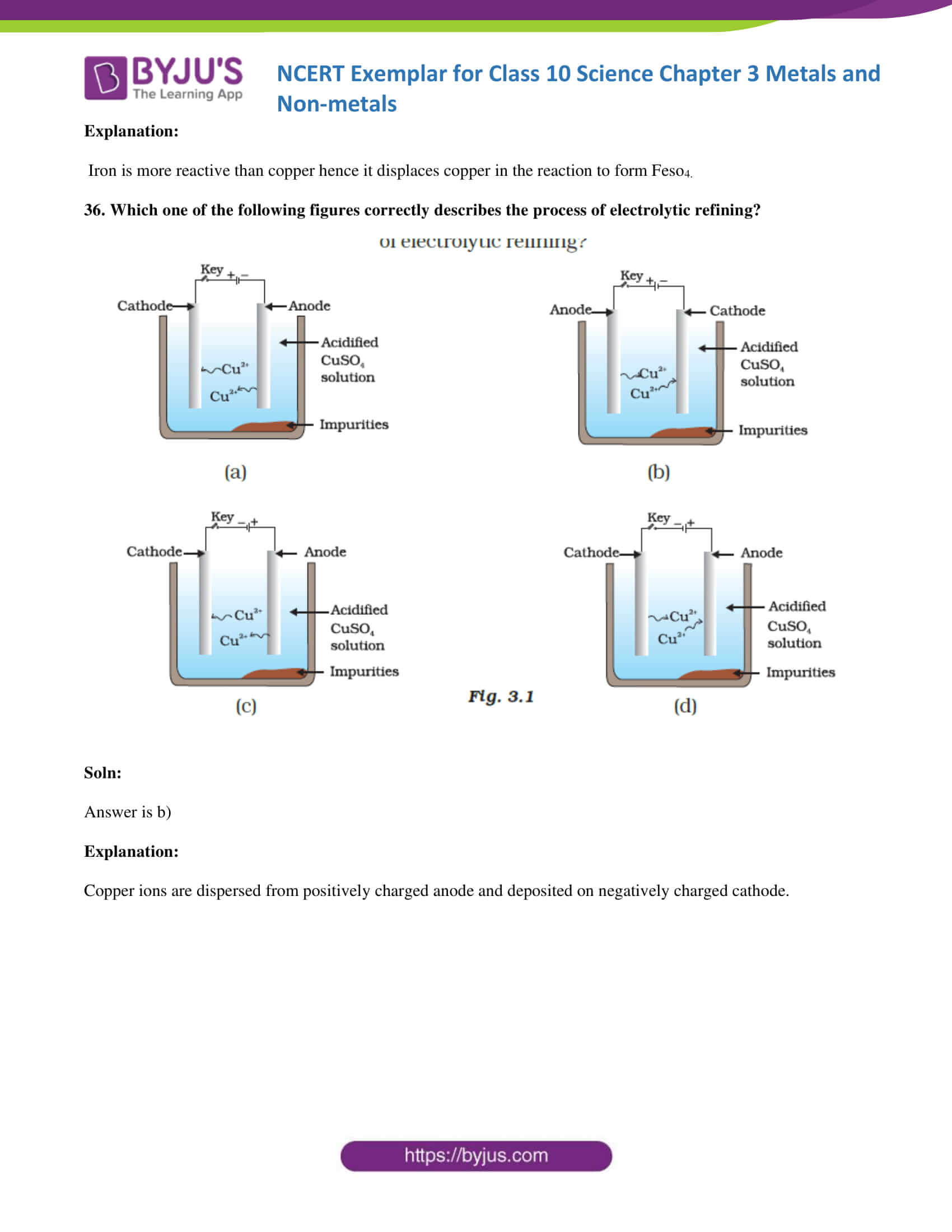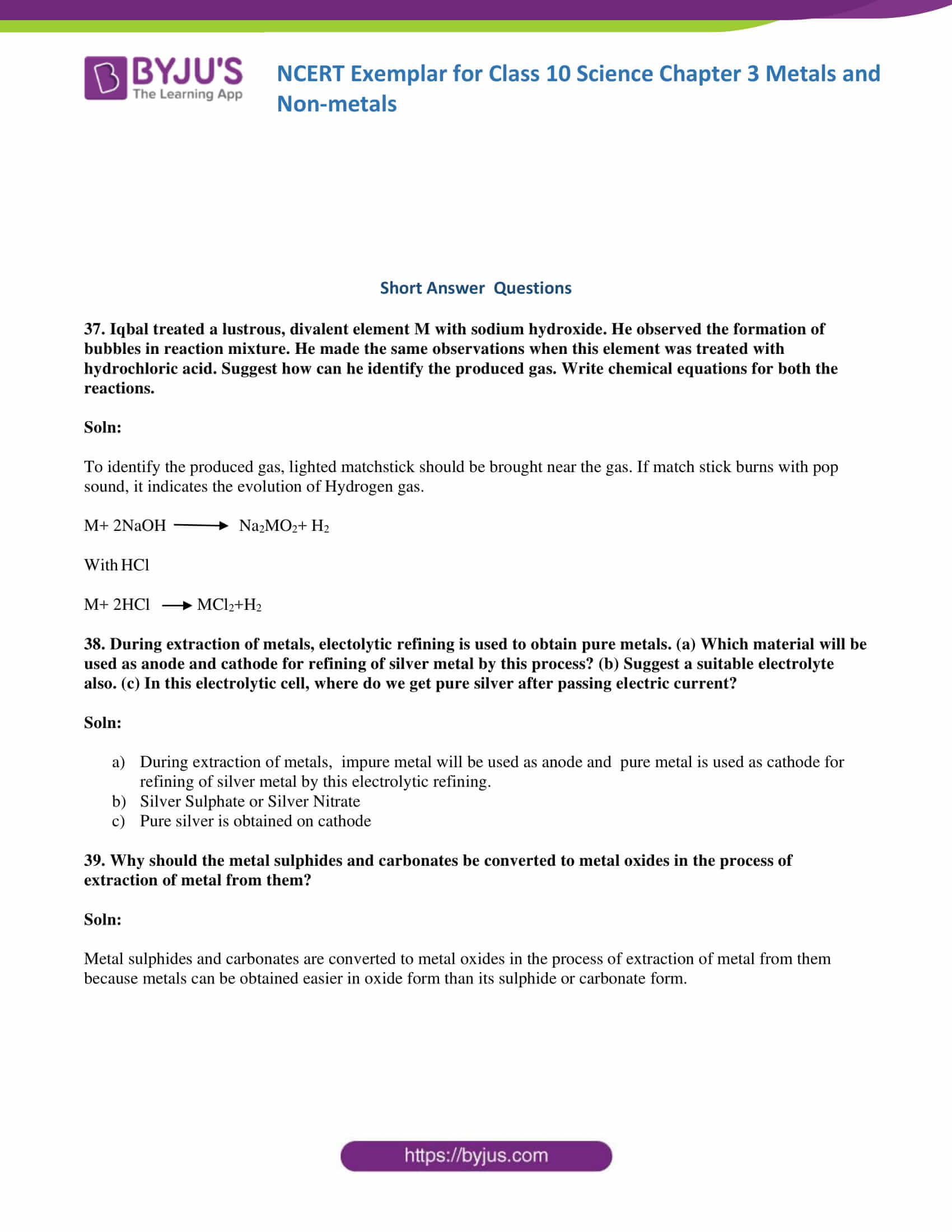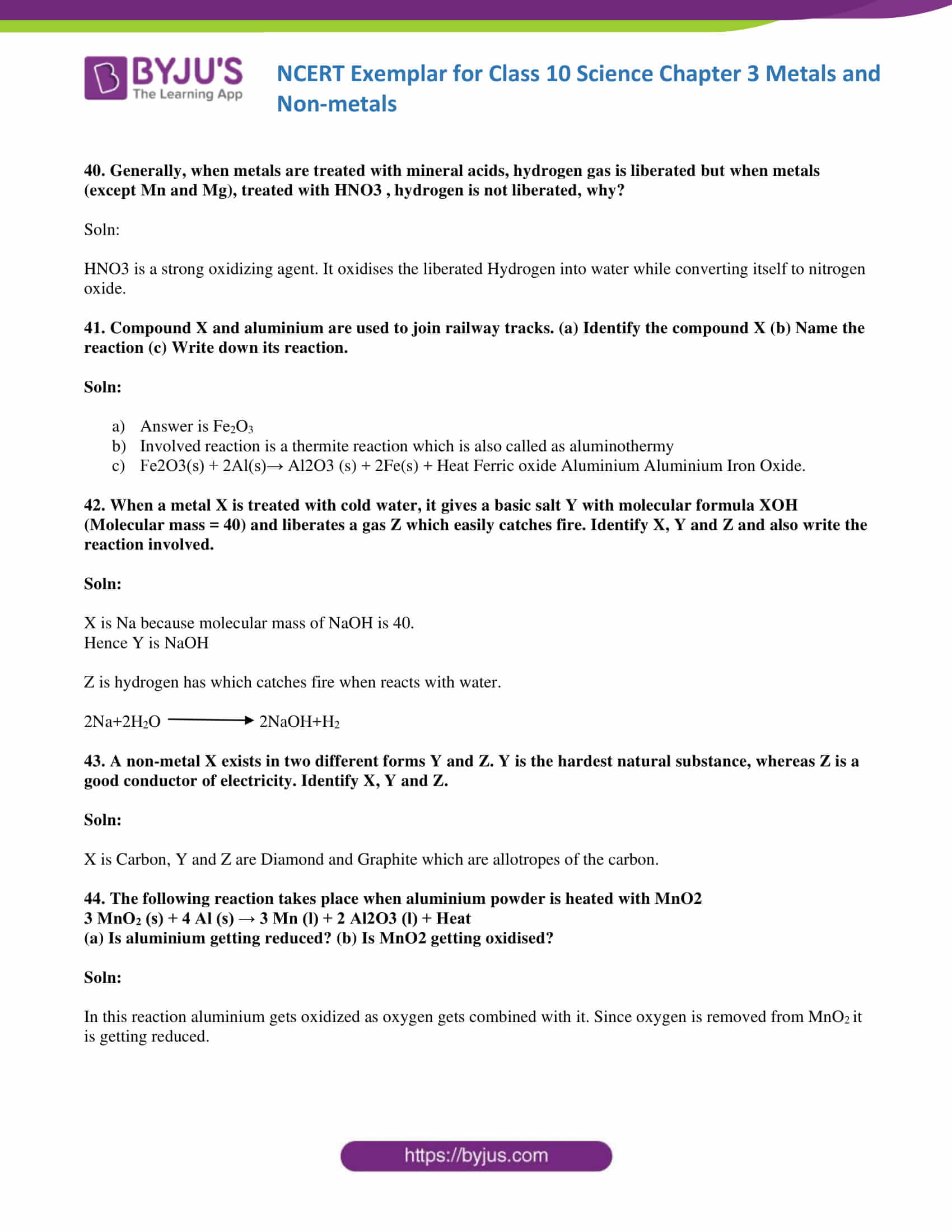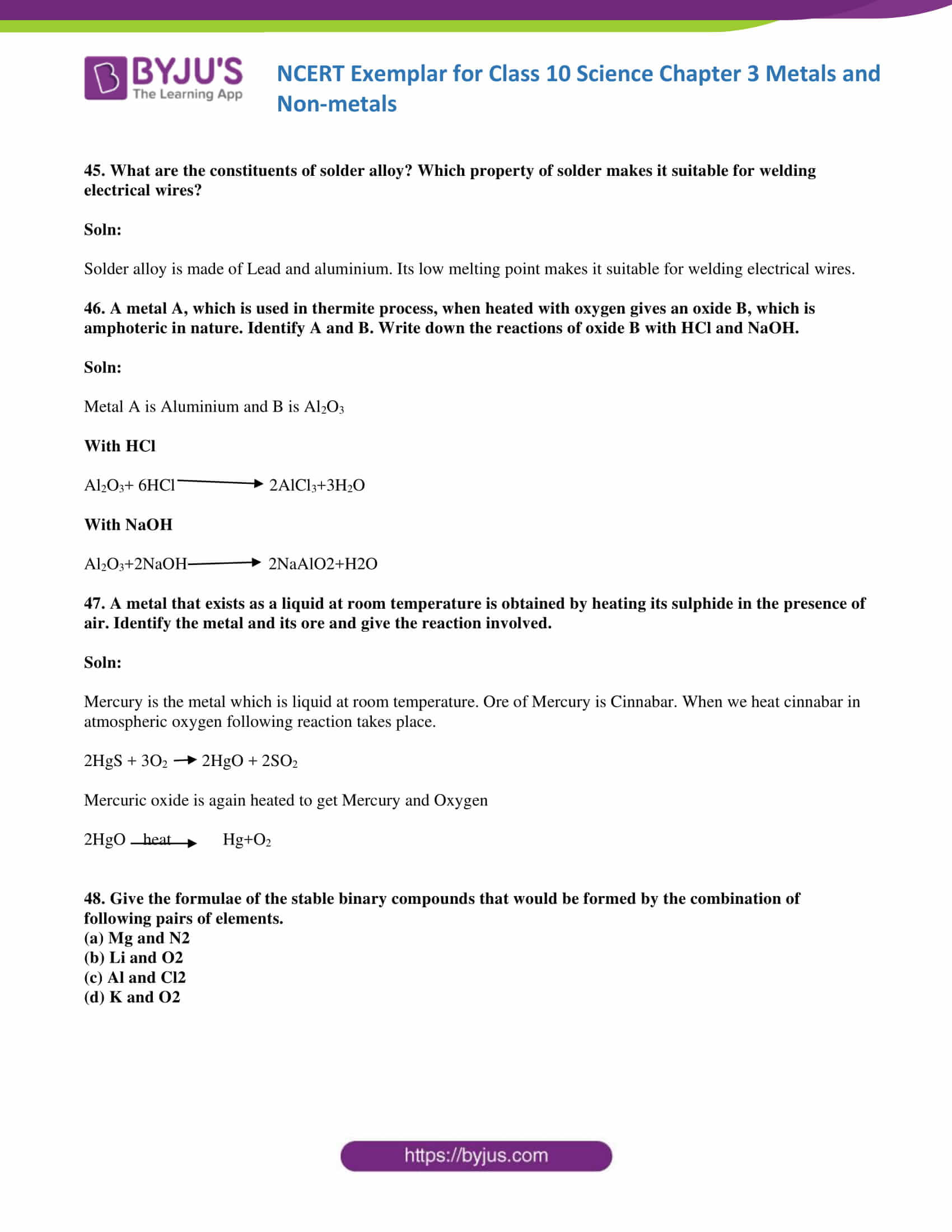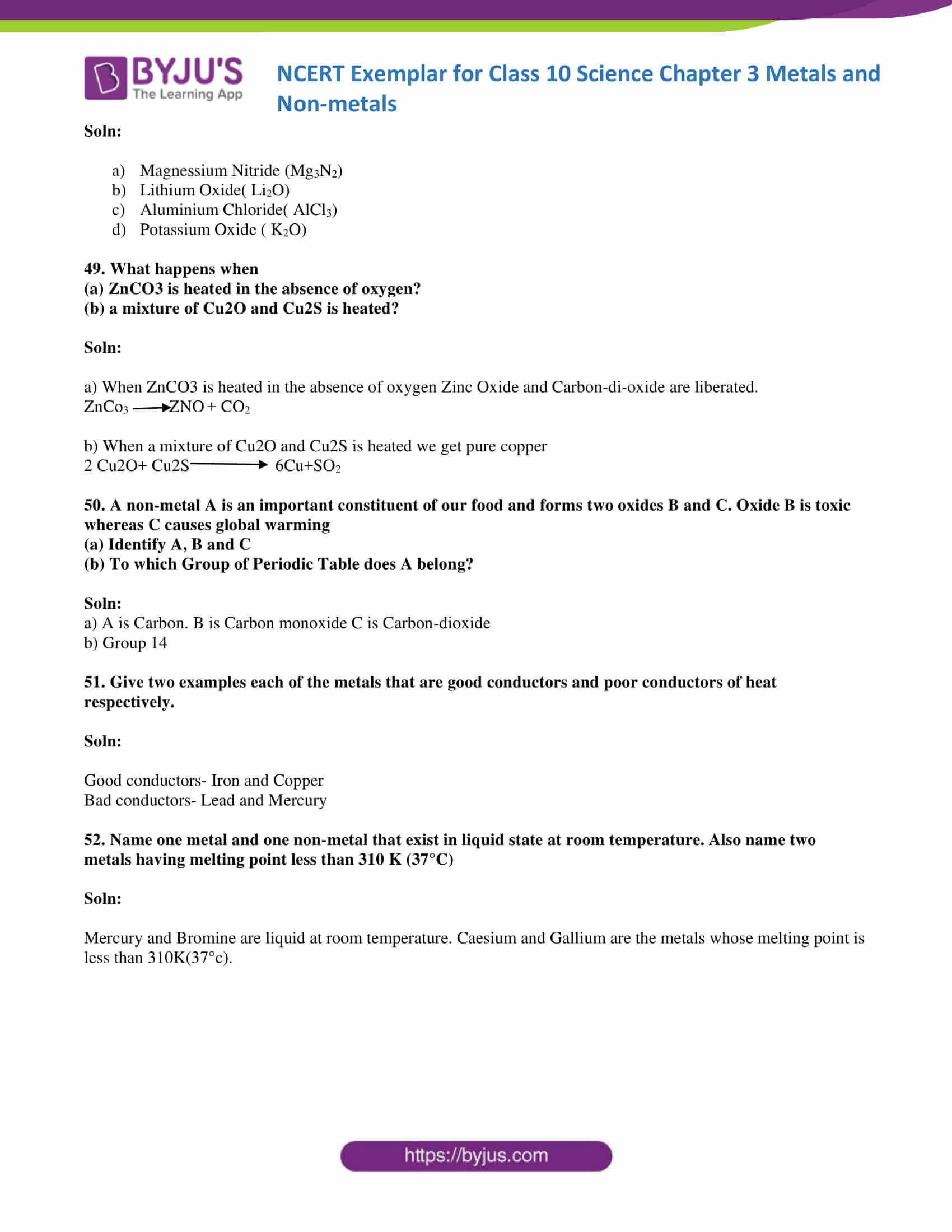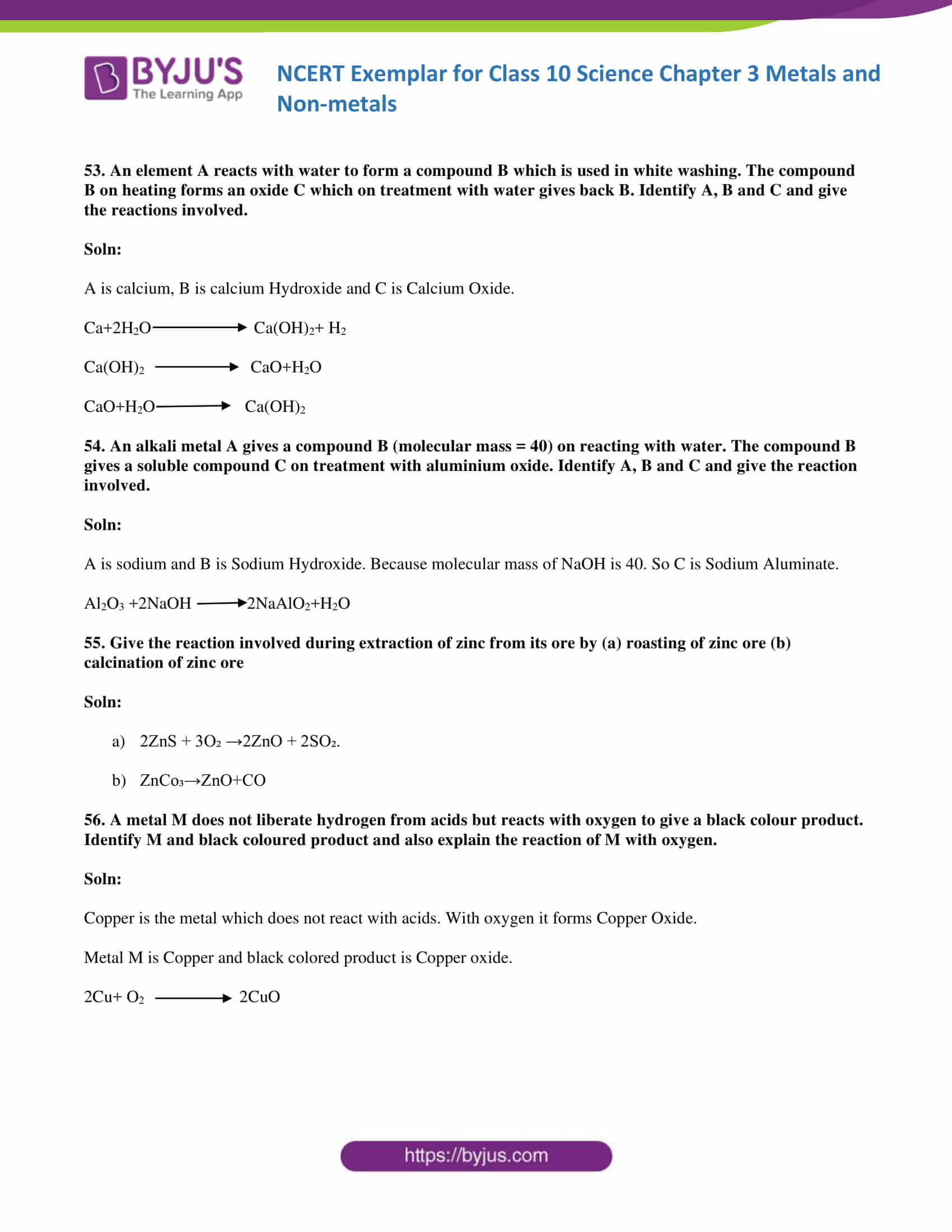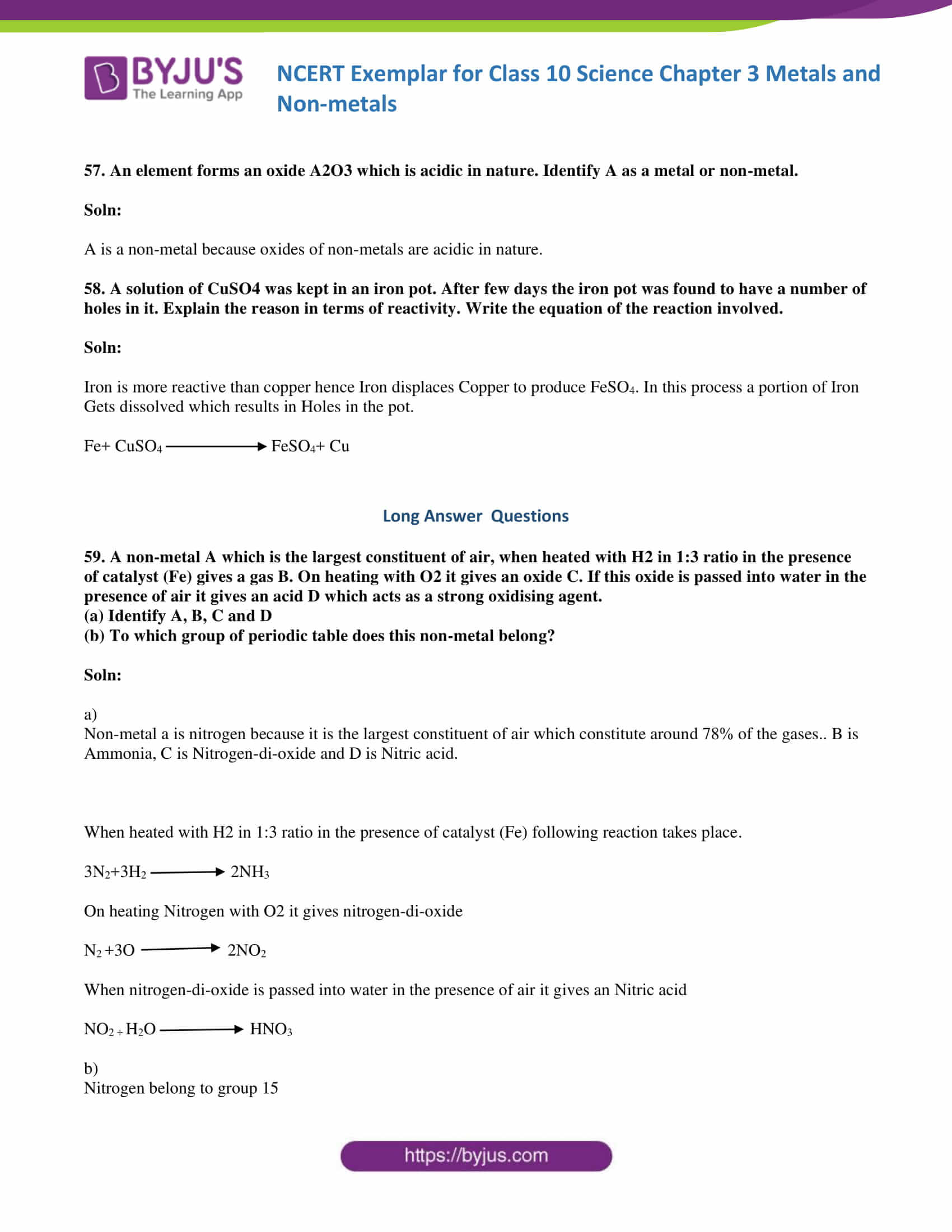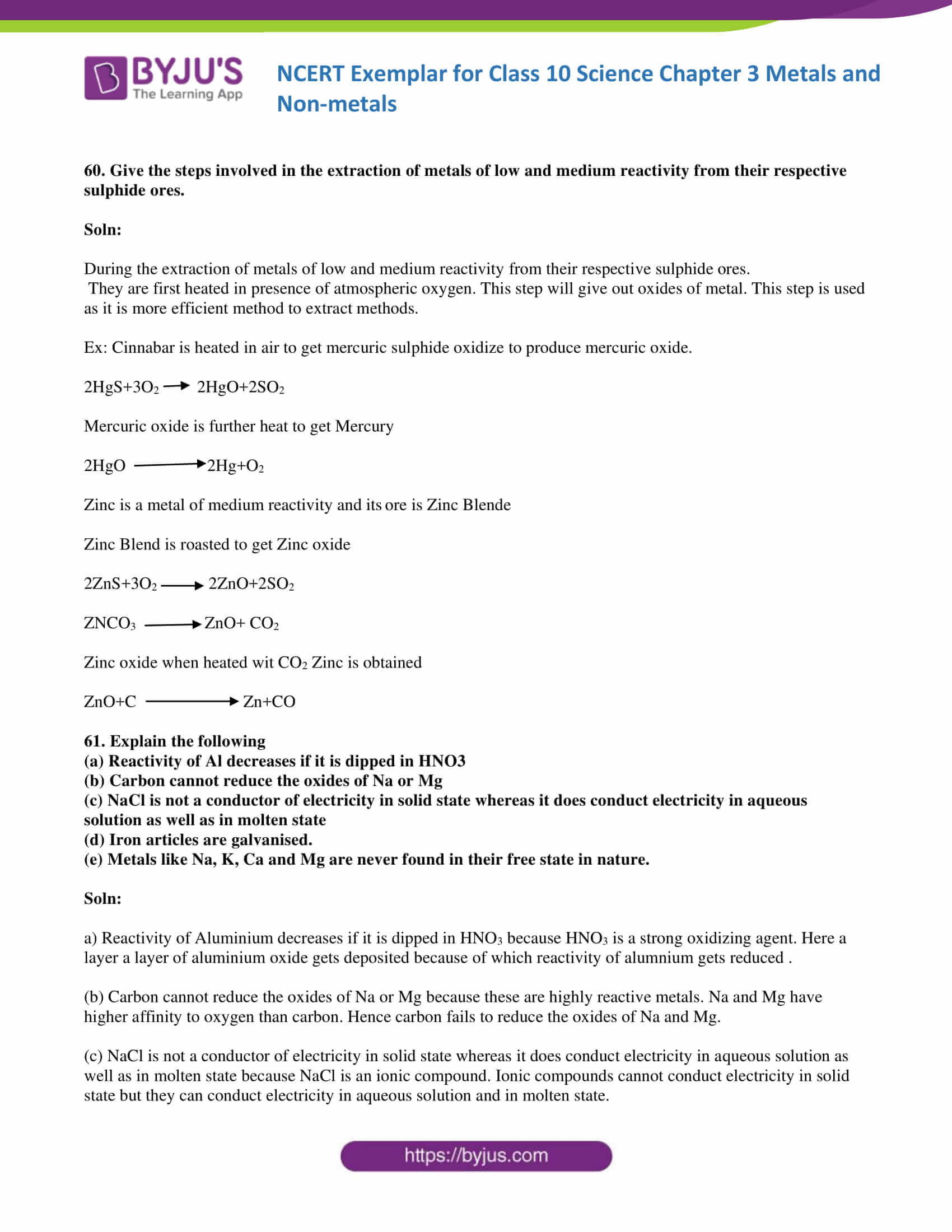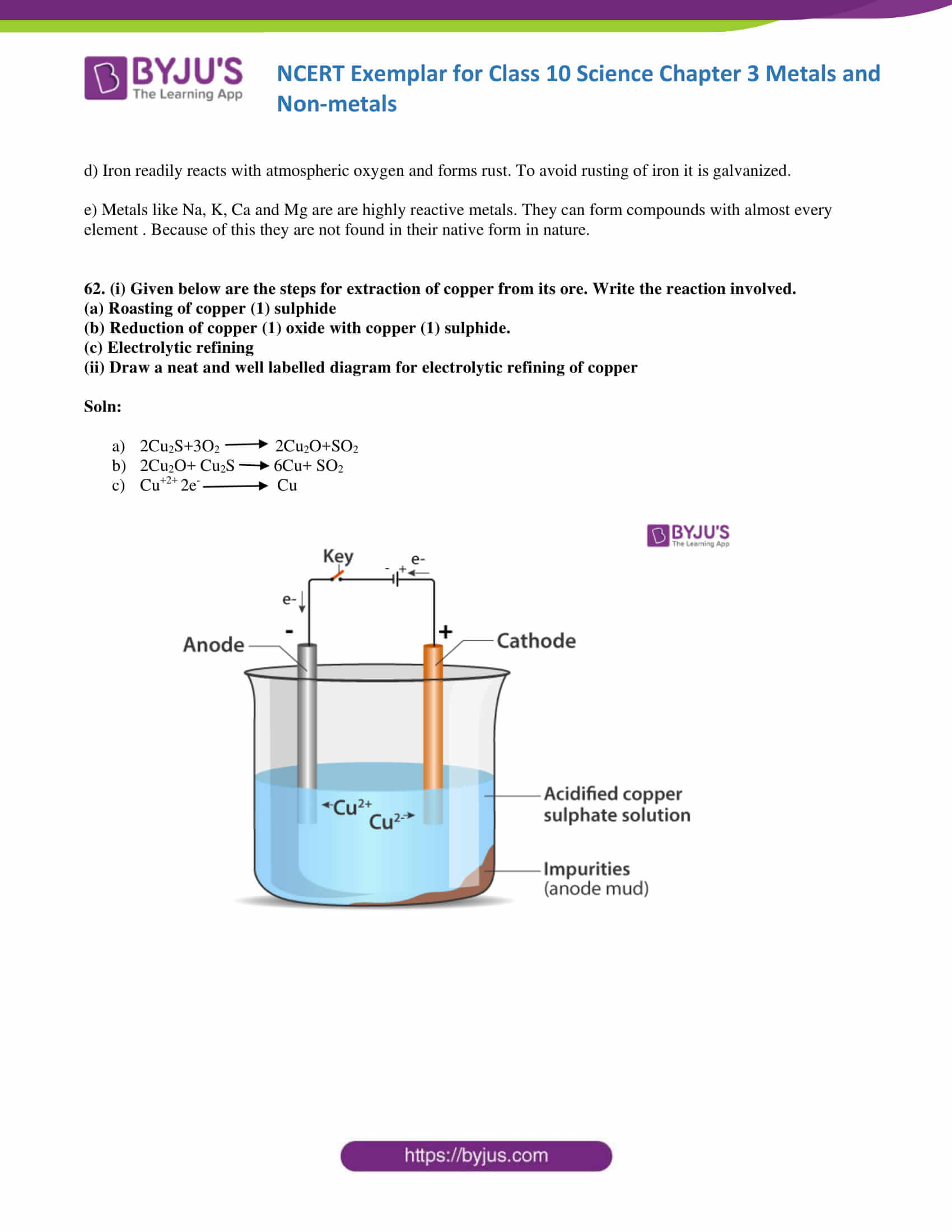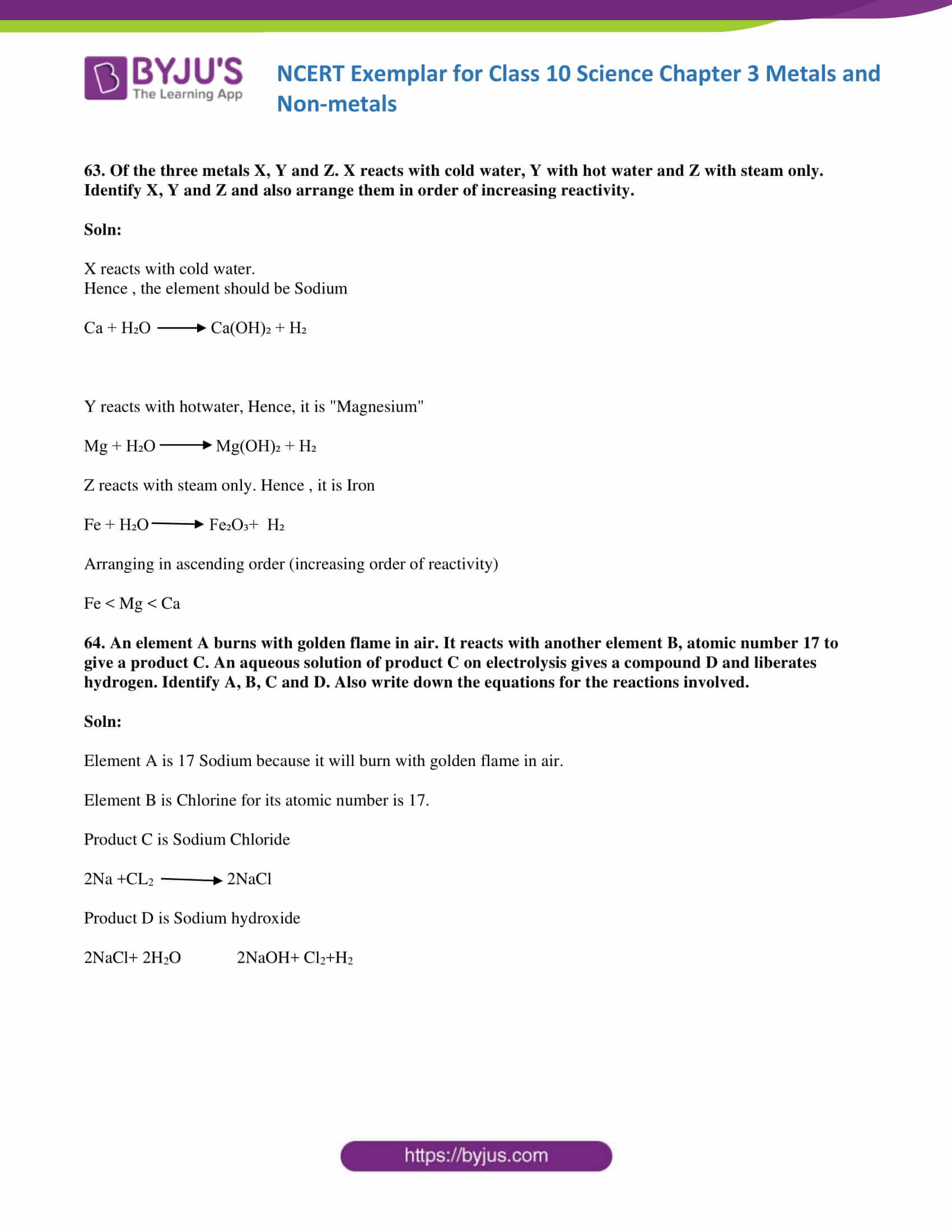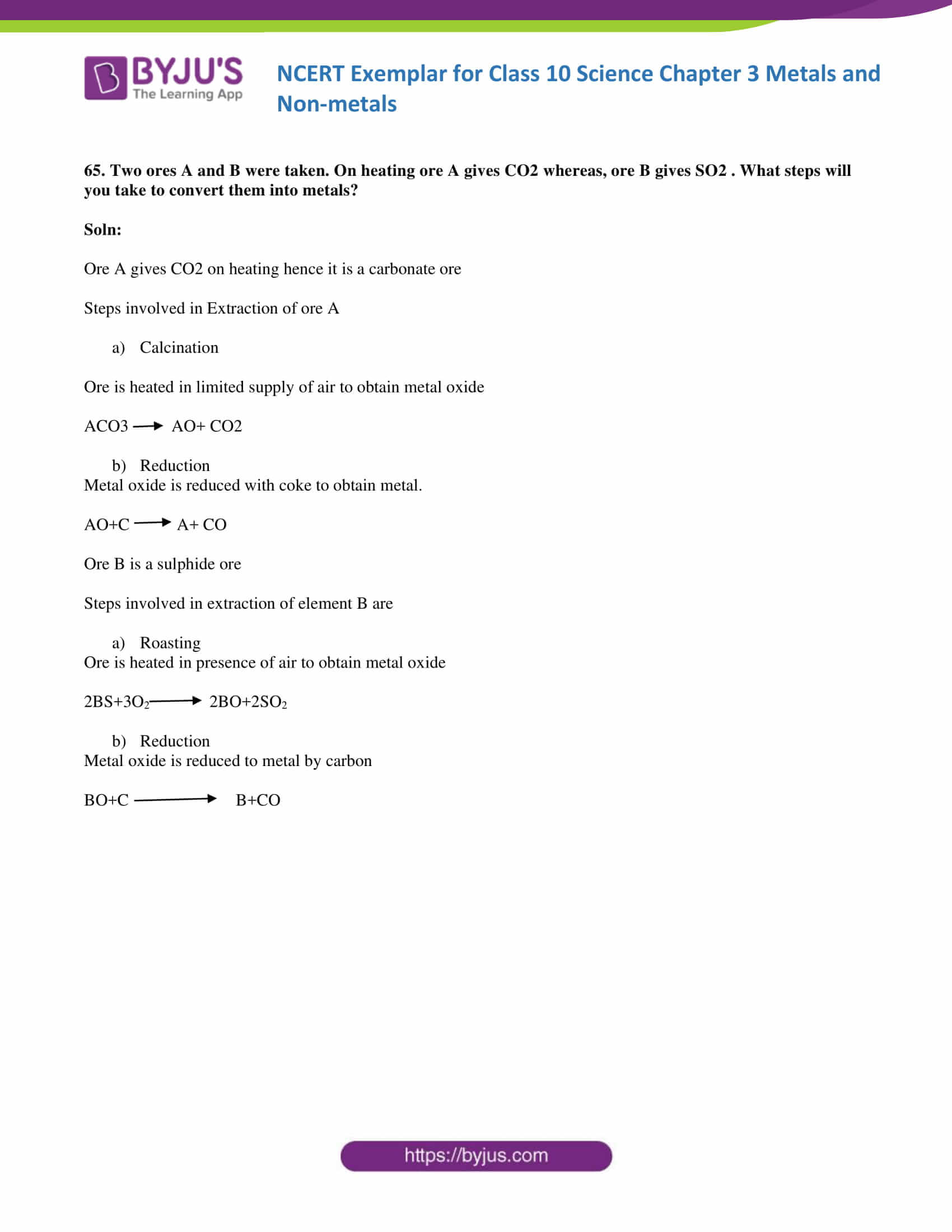### Multiple Choice Questions

1. Which of the following property is generally not shown by metals?

(a) Electrical conduction

(b) Sonorous in nature

(c) Dullness

(d) Ductility

Soln:

2. The ability of metals to be drawn into thin wires is known as

(a) ductility

(b) malleability

(c) sonorousity

(d) conductivity

Soln:

Explanation:

• Ductility is the property of metals to be drawn into thin wire.
• Malleability is property of metals to be drawn into a thin wire
• Sonorousity is the ability of a metal to be sonorous in nature, which means it is capable of making a deep, ringing sound.
• Conductivity is the ability to conduct electricity

3. Aluminium is used for making cooking utensils. Which of the following properties of aluminium are responsible for the same?

(i) Good thermal conductivity

(ii) Good electrical conductivity

(iii) Ductility

(iv) High melting point

(a) (i) and (ii)

(b) (i) and (iii)

(c) (ii) and (iii)

(d) (i) and (iv)

Soln:

The answer is (d) (i) and (iv)

Explanation:

Ductility and electric conductivity have no relation to cooking hence thermal conductivity, and high melting point are the right answers.

4. Which one of the following metals does not react with cold as well as hot water?

(a) Na

(b) Ca

(c) Mg

(d) Fe

Soln:

Explanation:

Sodium and Calcium react vigorously with water and Magnesium reacts with hot water to form Magnesium Oxide. Iron does not react with cold and hot water, but it reacts with steam.

5. Which of the following oxide(s) of iron would be obtained on the prolonged reaction of iron with steam?

(a) FeO

(b) Fe2O3

(c) Fe3O4

(d) Fe2O3 and Fe3O4

Soln:

Explanation:

3Fe+4H2O →Fe3O4+4H2

6. What happens when calcium is treated with water?

(i) It does not react with water

(ii) It reacts violently with water

(iii) It reacts less violently with water

(iv) Bubbles of hydrogen gas formed stick to the surface of calcium

(a) (i) and (iv)

(b) (ii) and (iii)

(c) (i) and (ii)

(d) (iii) and (iv)

Soln:

The answer is (d) (iii) and (iv)

Explanation:

Calcium reacts vigorously with water and forms hydrogen, which will make calcium to float.

7. Generally, metals react with acids to give salt and hydrogen gas. Which of the following acids does not give hydrogen gas on reacting with metals (except Mn and Mg)?

(a) H2 SO4

(b) HCl

(c) HNO3

(d) All of these

Soln:

Explanation:

Nitric acid is a powerful oxidizing agent. It reacts with the metal to form water.

8. The composition of aqua-regia is

(a) Dil.HCl: Conc. HNO3 3: 1

(b) Conc.HCl: Dil. HNO3 3 : 1

(c) Conc.HCl: Conc.HNO3 3 : 1

(d) Dil.HCl: Dil.HNO3 3: 1

Soln:

The answer is (c) Conc.HCl: Conc.HNO3 3: 1

9. Which of the following are not ionic compounds?

(i) KCl

(ii) HCl

(iii) CCl4

(iv) NaCl

(a) (i) and (ii)

(b) (ii) and (iii)

(c) (iii) and (iv)

(d) (i) and (iii)

Soln:

The answer is (b) (ii) and (iii)

Explanation:

HCl and CCl4 are covalent compounds; hence they cannot be ionic.

10. Which one of the following properties is not generally exhibited by ionic compounds?

(a) Solubility in water

(b) Electrical conductivity in solid-state

(c) High melting and boiling points

(d) Electrical conductivity in a molten state

Soln:

The answer is (b) Electrical conductivity in solid-state

Explanation:

In an ionic compound, free ions are not available in solid-state hence solid ionic compounds cannot conduct electricity.

11. Which of the following metals exist in their native state in nature?

1. Cu
2. Au
3. Zn
4. Ag

(a) (i) and (ii)

(b) (ii) and (iii)

(c) (ii) and (iv)

(d) (iii) and (iv)

Soln:

The answer is (c) (ii) and (iv)

Explanation:

Gold and silver are non-reactive metals because of they are non-reactive they exist in the native state in nature.

12. Metals are refined by using different methods. Which of the following metals are refined by electrolytic refining?

(i) Au

(ii) Cu

(iii) Na

(iv) K

(a) (i) and (ii)

(b) (i) and (iii)

(c) (ii) and (iii)

(d) (iii) and (iv)

Soln:

The answer is (d) (iii) and (iv)

Explanation:

Sodium and potassium are at the top in reactivity series hence they can be refined by electrolytic refining.

13. Silver articles become black on prolonged exposure to air. This is due to the formation of

(a) Ag3N

(b) Ag2O

(c) Ag2 S

(d) Ag2S and Ag3N

Soln:

Explanation:

Silver metal reacts with sulphur present in the atmosphere to form Ag2S. Ag2S is responsible for black colouration of silver articles.

14. Galvanisation is a method of protecting iron from rusting by coating with a thin layer of

(a) Gallium

(b) Aluminium

(c) Zinc

(d) Silver

Soln:

Explanation:

Galvanization is a process of applying a layer of Zinc on iron by using electrolysis.

15. Stainless steel is a very useful material for our life. In stainless steel, iron is mixed with

(a) Ni and Cr

(b) Cu and Cr

(c) Ni and Cu

(d) Cu and Au

Soln:

The answer is (a) Ni and Cr

Explanation :

Stainless steel is an alloy of Iron, Nicker and chromium. This alloy makes the metal strong, durable and corrosion-resistant.

16. If copper is kept open in the air, it slowly loses its shining brown surface and gains a green coating. It is due to the formation of

(a) CuSO4

(b) CuCO3

(c) Cu(NO3 ) 2

(d) CuO

Soln:

Explanation:

On exposure to air, copper reacts to atmospheric oxygen to form copper oxide layer which is green in colour.

17. Generally, metals are solid in nature. Which one of the following metals is found in liquid state at room temperature?

(a) Na

(b) Fe

(c) Cr

(d) Hg

Soln:

18. Which of the following metals are obtained by electrolysis of their chlorides in molten state?

(i) Na

(ii) Ca

(iii) Fe

(iv) Cu

(a) (i) and (iv)

(b) (iii) and (iv)

(c) (i) and (iii)

(d) (i) and (ii)

Soln:

The answer is (d) (i) and (ii)

Explanation:

Sodium and Calcium are in the top in reactivity series

19. Generally, non-metals are not lustrous. Which of the following nonmetal is lustrous?

(a) Sulphur

(b) Oxygen

(c) Nitrogen

(d) Iodine

Soln:

20. Which one of the following four metals would be displaced from the solution of its salts by the other three metals?

(a) Mg

(b) Ag

(c) Zn

(d) Cu

Soln:

Explanation:

Because silver is a non-reactive metal it can be displaced easily.

21. 2 mL each of concentrated HCl, HNO3 and a mixture of concentrated HCl and concentrated HNO3 in the ratio of 3: 1 were taken in test tubes labelled as A, B and C. A small piece of metal was put in each test tube. No change occurred in test tubes A and B but the metal got dissolved in test tube C respectively. The metal could be

(a) Al

(b) Au

(c) Cu

(d) Pt

Soln:

Explanation:

Answer is gold because solution C is a mixture of Concentrated HCL and Concentrated HNO3 which is called aqua regia. Aqua Regia dissolves gold.

22. An alloy is

(a) an element

(b) a compound

(c) a homogeneous mixture

(d) a heterogeneous mixture

Soln:

The answer is (c) a homogeneous mixture

Explanation:

An Alloy is a homogeneous mixture because its composition is uniform.

23. An electrolytic cell consists of

(i) positively charged cathode

(ii) negatively charged anode

(iii) positively charged anode

(iv) negatively charged cathode

(a) (i) and (ii)

(b) (iii) and (iv)

(c) (i) and (iii)

Soln:

The answer is (b) (iii) and (iv)

24. During electrolytic refining of zinc, it gets

(a) deposited on cathode

(b) deposited on anode

(c) deposited on the cathode as well as anode

(d) remains in the solution

Soln:

The answer is (a) deposited on cathode

Explanation:

Zinc is positively charged hence it moves towards the negatively charged cathode.

25. An element A is soft and can be cut with a knife. This is very reactive to air and cannot be kept open in the air. It reacts vigorously with water. Identify the element from the following

(a) Mg

(b) Na

(c) P

(d) Ca

Soln:

Explanation:

Sodium is soft which can be cut with a knife. Sodium reacts with water and air vigorously hence it is kept in kerosene.

26. Alloys are homogeneous mixtures of a metal with a metal or nonmetal. Which among the following alloys contain non-metal as one of its constituents?

(a) Brass

(b) Bronze

(c) Amalgam

(d) Steel

Soln:

Explanation:

Steel is an alloy made up of carbon and iron where carbon is a non-metal.

27. Which among the following statements is incorrect for magnesium metal?

(a) It burns in oxygen with a dazzling white flame

(b) It reacts with cold water to form magnesium oxide and evolves hydrogen gas

(c) It reacts with hot water to form magnesium hydroxide and evolves hydrogen gas

(d) It reacts with steam to form magnesium hydroxide and evolves hydrogen gas

Soln:

The answer is (b) It reacts with cold water to form magnesium oxide and evolves hydrogen gas

Explanation:

Magnesium reacts with cold water to form magnesium oxide and evolves hydrogen gas is a wrong statement because Magnesium Hydroxide is formed not Magnesium oxide.

28. Which among the following alloys contain mercury as one of its constituents?

(a) Stainless steel

(b) Alnico

(c) Solder

(d) Zinc amalgam

Soln:

The answer is (d) Zinc amalgam

Explanation:

Zinc amalgam is an alloy of Mercury and Zinc

29. Reaction between X and Y, forms compound Z. X loses electron and Y gains electron. Which of the following properties is not shown by Z?

(a) Has a high melting point

(b) Has a low melting point

(c) Conducts electricity in molten state

(d) Occurs as solid

Soln:

The answer is (b) Has a low melting point

Explanation:

Here Z is an ionic compound and Ionic compounds have melting point hence option b) is a wrong statement.

30. The electronic configurations of three elements X, Y and Z are X — 2, 8; Y — 2, 8, 7 and Z — 2, 8, 2. Which of the following is correct?

(a) X is a metal

(b) Y is a metal

(c) Z is a non-metal

(d) Y is a non-metal and Z is a metal

Soln:

The answer is (d) Y is a non-metal and Z is a metal

Explanation:

Element Y has 3 electron in its outer which is electronegative in nature. Z has 2 electrons in its outermost shell hence it is electropositive. Hence it is a metal.

31. Although metals form basic oxides, which of the following metals form an amphoteric oxide?

(a) Na

(b) Ca

(c) Al

(d) Cu

Soln:

Explanation:

Oxides of Aluminum are both acidic and basic in nature. Hence aluminium oxides are amphoteric in nature.

32. Generally, non-metals are not conductors of electricity. Which of the following is a good conductor of electricity?

(a) Diamond

(b) Graphite

(c) Sulphur

(d) Fullerene

Soln:

33. Electrical wires have a coating of an insulating material. The material, generally used is

(a) Sulphur

(b) Graphite

(c) PVC

(d) All can be used

Soln:

Explanation:

Sulphur is brittle in nature, hence it can be used and Graphite is a good conductor of electricity hence it cannot be used as an insulator.

34. Which of the following non-metals is a liquid?

(a) Carbon

(b) Bromine

(c) Phosphorus

(d) Sulphur

Soln:

35. Which of the following can undergo a chemical reaction?

(a) MgSO4 + Fe

(b) ZnSO4 + Fe

(c) MgSO4 + Pb

(d) CuSO4 + Fe

Soln:

The answer is (d) CuSO4 + Fe

Explanation:

Iron is more reactive than copper hence it displaces copper in the reaction to form Feso4.

36. Which one of the following figures correctly describes the process of electrolytic refining?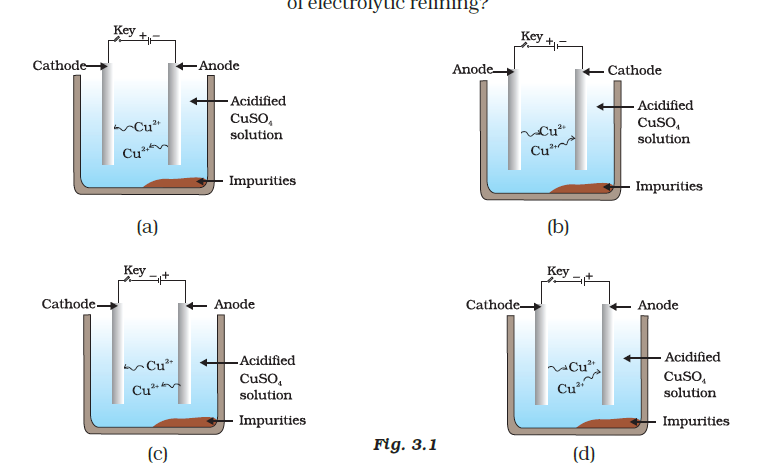Soln:

Explanation:

Copper ions are dispersed from the positively charged anode and deposited on the negatively charged cathode.

37. Iqbal treated a lustrous, divalent element M with sodium hydroxide. He observed the formation of bubbles in a reaction mixture. He made the same observations when this element was treated with hydrochloric acid. Suggest how can he identify the produced gas. Write chemical equations for both the reactions.

Soln:

To identify the produced gas, lighted matchstick should be brought near the gas. If match stick burns with a “pop” sound, it indicates the evolution of Hydrogen gas.

M+ 2NaOH → Na2MO2+ H2

With HCl

M+ 2HCl →MCl2+H2

38. During the extraction of metals, electrolytic refining is used to obtain pure metals. (a) Which material will be used as anode and cathode for refining of silver metal by this process? (b) Suggest a suitable electrolyte also. (c) In this electrolytic cell, where do we get pure silver after passing electric current?

Soln:

1. During extraction of metals, impure metal will be used as an anode, and pure metal is used as a cathode for refining of silver metal by this electrolytic refining.
2. Silver Sulphate or Silver Nitrate
3. Pure silver is obtained on the cathode

39. Why should the metal sulphides and carbonates be converted to metal oxides in the process of extraction of metal from them?

Soln:

Metal sulphides and carbonates are converted to metal oxides in the process of extraction of metal from them because metals can be obtained easier in oxide form than its sulphide or carbonate form.

40. Generally, when metals are treated with mineral acids, hydrogen gas is liberated, but when metals (except Mn and Mg), treated with HNO3, hydrogen is not liberated, why?

Soln:

HNO3 is a strong oxidizing agent. It oxidises the liberated Hydrogen into the water while converting itself to nitrogen oxide.

41. Compound X and aluminium are used to join railway tracks. (a) Identify the compound X (b) Name the reaction (c) Write down its reaction.

Soln:

2. The involved reaction is a thermite reaction which is also called as aluminothermy
3. Fe2O3(s) + 2Al(s)→ Al2O3 (s) + 2Fe(s) + Heat Ferric oxide Aluminium Aluminium Iron Oxide.

42. When a metal X is treated with cold water, it gives a basic salt Y with molecular formula XOH (Molecular mass = 40) and liberates a gas Z which easily catches fire. Identify X, Y and Z and also write the reaction involved.

Soln:

X is Na because the molecular mass of NaOH is 40.

Hence Y is NaOH

Z is hydrogen has which catches fire when reacts with water.

2Na+2H2O → 2NaOH+H2

43. A non-metal X exists in two different forms Y and Z. Y is the hardest natural substance, whereas Z is a good conductor of electricity. Identify X, Y and Z.

Soln:

X is Carbon, Y and Z are Diamond and Graphite, which are allotropes of the carbon.

44. The following reaction takes place when the aluminium powder is heated with MnO2

3 MnO2 (s) + 4 Al (s) → 3 Mn (l) + 2 Al2O3 (l) + Heat

(a) Is aluminium getting reduced? (b) Is MnO2 getting oxidised?

Soln:

In this reaction, aluminium gets oxidized as oxygen gets combined with it. Since oxygen is removed from MnO2, it is getting reduced.

45. What are the constituents of solder alloy? Which property of solder makes it suitable for welding electrical wires?

Soln:

Solder alloy is made of Lead and aluminium. Its low melting point makes it suitable for welding electrical wires.

46. A metal A, which is used in thermite process, when heated with oxygen gives an oxide B, which is amphoteric in nature. Identify A and B. Write down the reactions of oxide B with HCl and NaOH.

Soln:

Metal A is Aluminium and B is Al→2O3

With HCl

Al2O3+ 6HCl →2AlCl3+3H2O

With NaOH

Al2O3+2NaOH →2NaAlO2+H2O

47. A metal that exists as a liquid at room temperature is obtained by heating its sulphide in the presence of air. Identify the metal and its ore and give the reaction involved.

Soln:

Mercury is the metal which is liquid at room temperature. Ore of Mercury is Cinnabar. When we heat cinnabar in atmospheric oxygen following reaction takes place.

2HgS + 3O2→ 2HgO + 2SO2

Mercuric oxide is again heated to get Mercury and Oxygen

2HgO

$$\begin{array}{l}\overset{heat}{\rightarrow}\end{array}$$
Hg+O2

48. Give the formulae of the stable binary compounds that would be formed by the combination of the following pairs of elements.

(a) Mg and N2

(b) Li and O2

(c) Al and Cl2

(d) K and O2

Soln:

1. Magnesium Nitride (Mg3N2)
2. Lithium Oxide( Li2O)
3. Aluminium Chloride( AlCl3)
4. Potassium Oxide ( K2O)

49. What happens when

(a) ZnCO3 is heated in the absence of oxygen?

(b) a mixture of Cu2O and Cu2S is heated?

Soln:

a) When ZnCO3 is heated in the absence of oxygen Zinc Oxide and Carbon-di-oxide are liberated.

ZnCo3 →ZnO + CO2

b) When a mixture of Cu2O and Cu2S is heated, we get pure copper

2 Cu2O+ Cu2S →6Cu+SO2

50. A non-metal A is an important constituent of our food and forms two oxides B and C. Oxide B is toxic whereas C causes global warming

(a) Identify A, B and C

(b) To which Group of Periodic Table does A belong?

Soln:

a) A is Carbon. B is Carbon monoxide and C is Carbon-dioxide

b) Group 14

51. Give two examples each: The metals that are good conductors and poor conductors of heat, respectively.

Soln:

Good conductors- Iron and Copper

52. Name one metal and one non-metal that exist in a liquid state at room temperature. Also, name two metals having a melting point less than 310 K (37°C)

Soln:

Mercury and Bromine are liquid at room temperature. Caesium and Gallium are the metals whose melting point is less than 310K(37°c).

53. An element A reacts with water to form a compound B which is used in whitewashing. The compound B on heating forms an oxide C which on treatment with water gives back B. Identify A, B and C and give the reactions involved.

Soln:

A is calcium, B is calcium Hydroxide, and C is Calcium Oxide.

Ca+2H2O →Ca(OH)2+ H2

Ca(OH)2 →CaO+H2O

CaO+H2O→ Ca(OH)2

54. An alkali metal A gives a compound B (molecular mass = 40) on reacting with water. The compound B gives a soluble compound C on treatment with aluminium oxide. Identify A, B and C and give the reaction involved.

Soln:

A is sodium and B is Sodium Hydroxide. Because the molecular mass of NaOH is 40. So C is Sodium Aluminate.

Al2O3 +2NaOH →2NaAlO2+H2O

55. Give the reaction involved during the extraction of zinc from its ore by (a) roasting of zinc ore (b) calcination of zinc ore

Soln:

1. 2ZnS + 3O2→2ZnO + 2SO2.
2. ZnCo3→ZnO+CO

56. A metal M does not liberate hydrogen from acids but reacts with oxygen to give a black colour product. Identify M and black coloured product and also explain the reaction of M with oxygen.

Soln:

Copper is the metal which does not react with acids. With oxygen, it forms Copper Oxide.

Metal M is Copper and black coloured product is copper oxide.

2Cu+ O2 →2CuO

57. An element forms an oxide A2O3 which is acidic in nature. Identify A as metal or non-metal.

Soln:

A is a non-metal because oxides of non-metals are acidic in nature.

58. A solution of CuSO4 was kept in an iron pot. After a few days, the iron pot was found to have a number of holes in it. Explain the reason in terms of reactivity. Write the equation of the reaction involved.

Soln:

Iron is more reactive than copper hence Iron displaces Copper to produce FeSO4. In this process, a portion of Iron Gets dissolved which results in Holes in the pot.

Fe+ CuSO4 →FeSO4+ Cu

59. A non-metal A which is the largest constituent of air, when heated with H2 in 1:3 ratio in the presence of a catalyst (Fe) gives a gas B. On heating with O2 it gives an oxide C. If this oxide is passed into water in the presence of air, it gives an acid D which acts as a strong oxidising agent.

(a) Identify A, B, C and D

(b) To which group of periodic table does this non-metal belong?

Soln:

a)Non-metal “A” is nitrogen because it is the largest constituent of air which constitute around 78% of the gases. B is Ammonia, C is Nitrogen-di-oxide and D is Nitric acid.

When heated with H2 in 1:3 ratio in the presence of a catalyst (Fe) following reaction takes place.

3N2+3H2 →2NH3

On heating Nitrogen with O2, it gives nitrogen-di-oxide

N2 +3O →2NO2

When nitrogen-dioxide is passed into the water in the presence of air, it gives Nitric acid

NO2 + H2O →HNO3

b)Nitrogen belongs to group 15

60. Give the steps involved in the extraction of metals of low and medium reactivity from their respective sulphide ores.

Soln:

During the extraction of metals of low and medium reactivity from their respective sulphide ores.

They are first heated in the presence of atmospheric oxygen. This step will give out oxides of metal. This step is used as it is a more efficient method to extract methods.

Ex: Cinnabar is heated in air to get mercuric sulphide to oxidize to produce mercuric oxide.

2HgS+3O2 →2HgO+2SO2

Mercuric oxide is further heat to get Mercury

2HgO→ 2Hg+O2

Zinc is a metal of medium reactivity, and its ore is Zinc Blende

Zinc Blend is roasted to get Zinc oxide

2ZnS+3O2 →2ZnO+2SO2

ZNCO3 →ZnO+ CO2

Zinc oxide when heated wit CO2 Zinc is obtained

ZnO+C→ Zn+CO

61. Explain the following

(a) Reactivity of Al decreases if it is dipped in HNO3

(b) Carbon cannot reduce the oxides of Na or Mg

(c) NaCl is not a conductor of electricity in the solid-state whereas it does conduct electricity in aqueous solution as well as in molten state

(d) Iron articles are galvanised.

(e) Metals like Na, K, Ca and Mg are never found in their free state in nature.

Soln:

a) Reactivity of Aluminium decreases if it is dipped in HNO3 because HNO3 is a strong oxidizing agent. Here, a layer of aluminium oxide gets deposited because of which reactivity of aluminium gets reduced.

(b) Carbon cannot reduce the oxides of Na or Mg because these are highly reactive metals. Na and Mg have a higher affinity to oxygen than carbon. Hence carbon fails to reduce the oxides of Na and Mg.

(c) NaCl is not a conductor of electricity in the solid-state whereas it does conduct electricity in aqueous solution as well as in molten state because NaCl is an ionic compound. Ionic compounds cannot conduct electricity in the solid-state, but they can conduct electricity in aqueous solution and in a molten state.

d) Iron readily reacts with atmospheric oxygen and forms rust. To avoid rusting of iron, it is galvanized.

e) Metals like Na, K, Ca and Mg are highly reactive metals. They can form compounds with almost every element. Because of this, they are not found in their native form in nature.

62. (i) Given below are the steps for extraction of copper from its ore. Write the reaction involved.

(a) Roasting of copper (1) sulphide

(b) Reduction of copper (1) oxide with copper (1) sulphide.

(c) Electrolytic refining

(ii) Draw a neat and well-labelled diagram for electrolytic refining of copper

Soln:

1. 2Cu2S+3O2 →2Cu2O+SO2
2. 2Cu2O+ Cu2S →6Cu+ SO2
3. Cu+2+ 2e→ Cu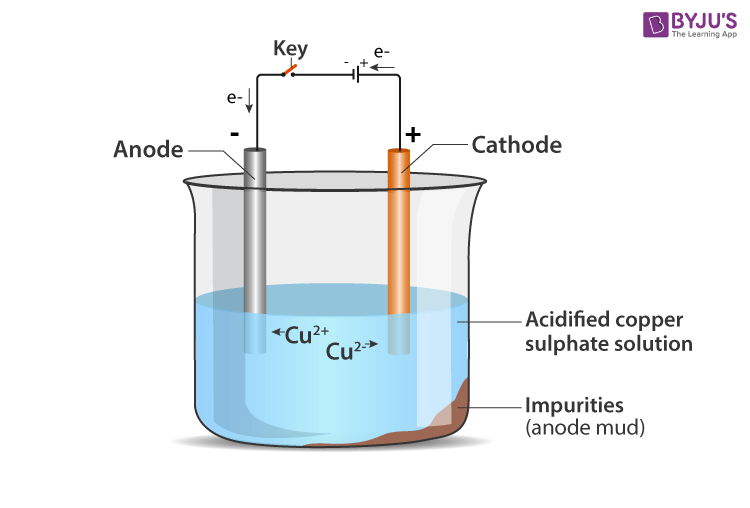63. Of the three metals X, Y and Z. X reacts with cold water, Y with hot water and Z with steam only. Identify X, Y and Z and also arrange them in order of increasing reactivity.

Soln:

X reacts with cold water.

Hence, the element should be Sodium

Na + H₂O → 2NaOH + H₂

Y reacts with hot water; hence, it is “Magnesium.”

Mg + H₂O →  Mg(OH)₂ + H₂

Z reacts with steam only. Hence, it is Iron

Fe + H₂O → Fe₂O₃+  H₂

Arranging in ascending order (increasing order of reactivity)

Fe < Mg < Ca

64. An element A burns with golden flame in the air. It reacts with another element B, atomic number 17 to give a product C. An aqueous solution of product C on electrolysis gives a compound D and liberates hydrogen. Identify A, B, C and D. Also write down the equations for the reactions involved.

Soln:

Element A is Sodium because it will burn with a golden flame in the air.

Element B is Chlorine for its atomic number is 17.

Product C is Sodium Chloride

2Na +CL2 →2NaCl

Product D is Sodium hydroxide

2NaCl+ 2H2O→ 2NaOH+ Cl2+H2

65. Two ores A and B were taken. On heating ore A gives CO2 whereas, ore B gives SO2. What steps will you take to convert them into metals?

Soln:

Ore A gives CO2 on heating hence it is a carbonate ore

Steps involved in Extraction of ore A

a) Calcination

Ore is heated in a limited supply of air to obtain a metal oxide

ACO3→ AO+ CO2

b)Reduction

The metal oxide is reduced with coke to obtain metal.

AO+C→ A+ CO

Ore B is a sulphide ore

Steps involved in the extraction of element B are

a) Roasting

Ore is heated in the presence of air to obtain a metal oxide

2BS+3O2→ 2BO+2SO2

b) Reduction

The metal oxide is reduced to metal by carbon

BO+C→ B+CO

### NCERT Exemplar Class 10 Science Chapter 3

Metals and non-metals Class 10 Exemplar provide you with necessary insights on the concepts involved and makes you ready to face different kinds of questions. This exemplar has Metals and Non-metals Class 10 important questions and answers, metals and non-metals Class 10 extra questions, question bank on metals and non-metals Class 10 and important question taken from the previous year question paper.

Important topics of Class 10 Metals and Non-metals

 Section Number Topic 3.1 Physical Properties 3.1.1 Metals 3.1.2 Non-metals 3.2 Chemical Properties Of Metals 3.2.1 What Happens When Metals Are Burnt In Air? 3.2.2 What Happens When Metals React With Water? 3.2.3 What Happens When Metals React With Acids? 3.2.4 How Do Metals React With Solutions Of Other Metal Salts?

The schools affiliated with CBSE are advised to follow the NCERT curriculum and textbooks. NCERT textbooks are one of the best study materials for the students of Class 10 NCERT with all topics covered in the CBSE syllabus (updated 2021-2022).

To score good marks in the examination; students should solve the previous year question papers and sample papers, solving the previous year question papers will help the students to determine the difficulty level of the questions and the marking scheme. For comprehensive learning opportunity, visit BYJU’S website or download BYJU’S learning App.

## Frequently Asked Questions on NCERT Exemplar Solutions for Class 10 Science Chapter 3

### Mention the topics included in Chapter 3 of NCERT Exemplar Solutions for Class 10 Science.

The topics included in the Chapter 3 of NCERT Exemplar Solutions for Class 10 Science are –
3.1 Physical Properties
3.1.1 Metals
3.1.2 Non-metals
3.2 Chemical Properties Of Metals
3.2.1 What Happens When Metals Are Burnt In Air?
3.2.2 What Happens When Metals React With Water?
3.2.3 What Happens When Metals React With Acids?
3.2.4 How Do Metals React With Solutions Of Other Metal Salts?

### How to balance the chemical equations present in Chapter 3 of NCERT Exemplar Solutions for Class 10 Science faster?

Students of Class 10 should learn to write chemical equations present in the NCERT textbook to get an idea about the important equations present in them. If doubts arise while writing the equations in the exercise problems, students can refer to the NCERT Exemplar Solutions for Class 10 Science from BYJU’S where each and every question is solved accurately. The solutions contain explanations for each step to help students understand the method of writing the chemical equations without any difficulty.

### What are the key features of NCERT Exemplar Solutions for Class 10 Science Chapter 3?

The key features of NCERT Exemplar Solutions for Class 10 Science Chapter 3 are –
1. The answers are prepared by highly experienced science experts.
2. The solutions are accurate without any error based on the latest CBSE syllabus.
3. Every important topic is explained in a simple language to help students score well in the exams.
4. Students get a thorough understanding of the important concepts using the NCERT Exemplar Solutions from BYJU’S.